# Refraction Through a Lens

## Exercise 5(A)

#### Question 1

What is a lens?

A lens is a transparent refracting medium bounded by either two spherical surfaces or one surface spherical and another surface plane.

There are two types of lenses —

1. Convex or converging lens
2. Concave or diverging lens

#### Question 2

Name the two kinds of lens? Draw diagrams to illustrate them.

Two types of lenses are —

1. Convex or converging lens
2. Concave or diverging lens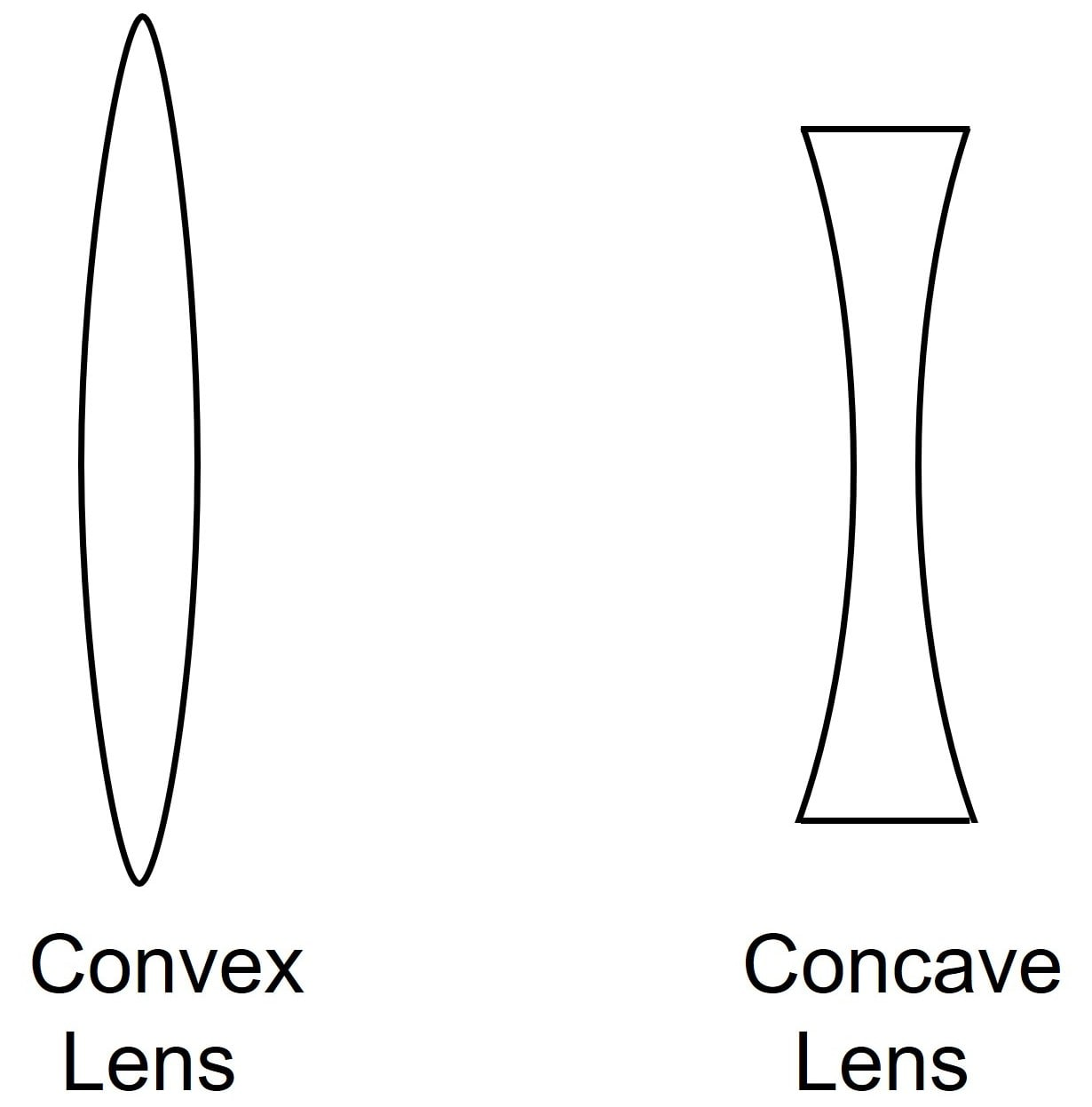#### Question 3

State difference between a convex and a concave lens in their —

(a) appearance, and

(b) action on the incident light.

(a) The difference between a convex and a concave lens in terms of appearance is as follows —

Convex lensConcave lens
A convex lens is thick in the middle and thin at its periphery.A concave lens is thin in the middle and thick at its periphery.
It bulges out in the middle.It is bent inwards in the middle.

(b) The difference between a convex and a concave lens in terms of action on the incident light is as follows —

Convex lensConcave lens
A convex lens converges the incident rays towards the principal axis.A concave lens diverges the incident rays away from the principal axis.

#### Question 4

Which lens is converging —

(i) an equiconcave lens or an equiconvex lens?

(ii) a concavo-convex lens or a convexo-concave lens?

(i) An equiconvex lens or bi convex has both its surfaces convex, and is a converging lens.

(ii) A concavo-convex lens is thicker in the middle and thinner at the edges and has a converging action on a light beam. Hence, a concavo-convex lens is a converging lens.

#### Question 5

Out of the two lenses, one concave and the other convex, state which one will show the divergent action on a light beam. Draw diagrams to illustrate your answer.

A concave lens is thick at its periphery and thin in the middle. In other words, a lens which is bent inwards in the middle, is the concave lens. Such a lens diverges the light rays incident on it, so it is called a diverging lens.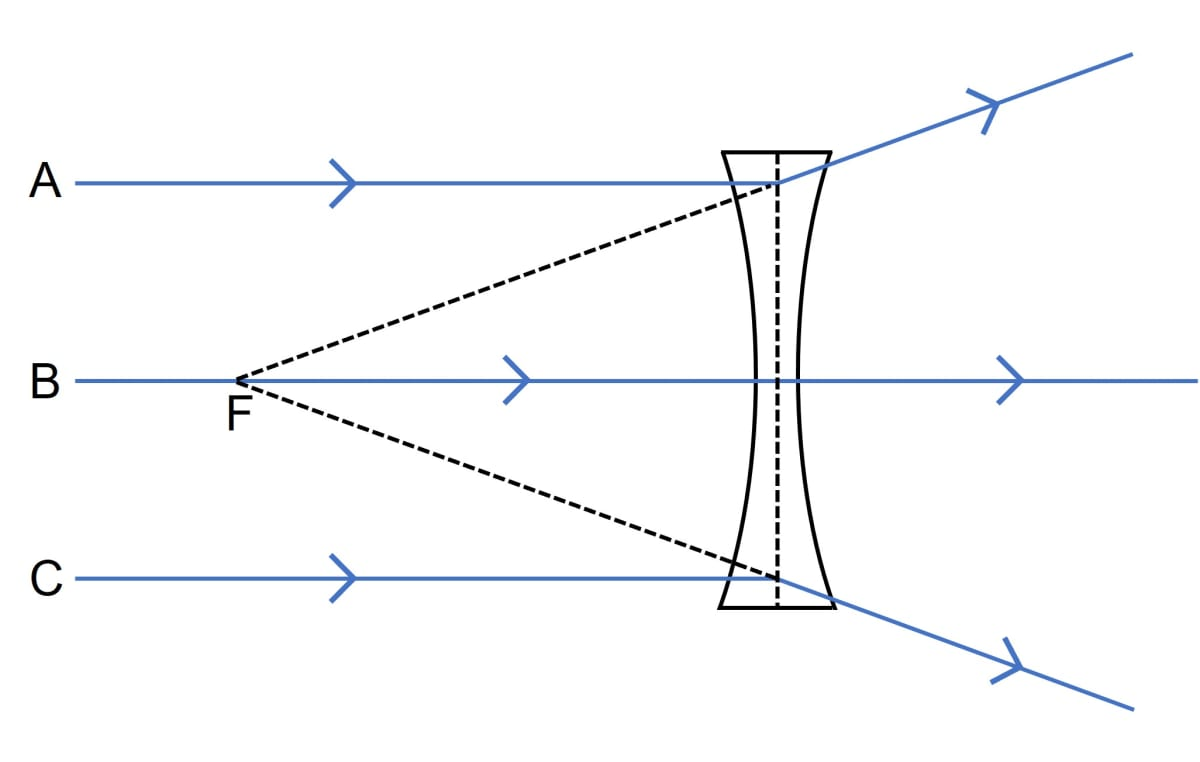#### Question 6

Show by a diagram the refraction of two light rays incident parallel to the principal axis on a convex lens by treating it as a combination of a glass slab and two triangular glass prisms.

Below diagram shows the refraction of two light rays incident parallel to the principal axis on a convex lens. The convex lens is shown as a combination of a glass slab and two triangular glass prisms: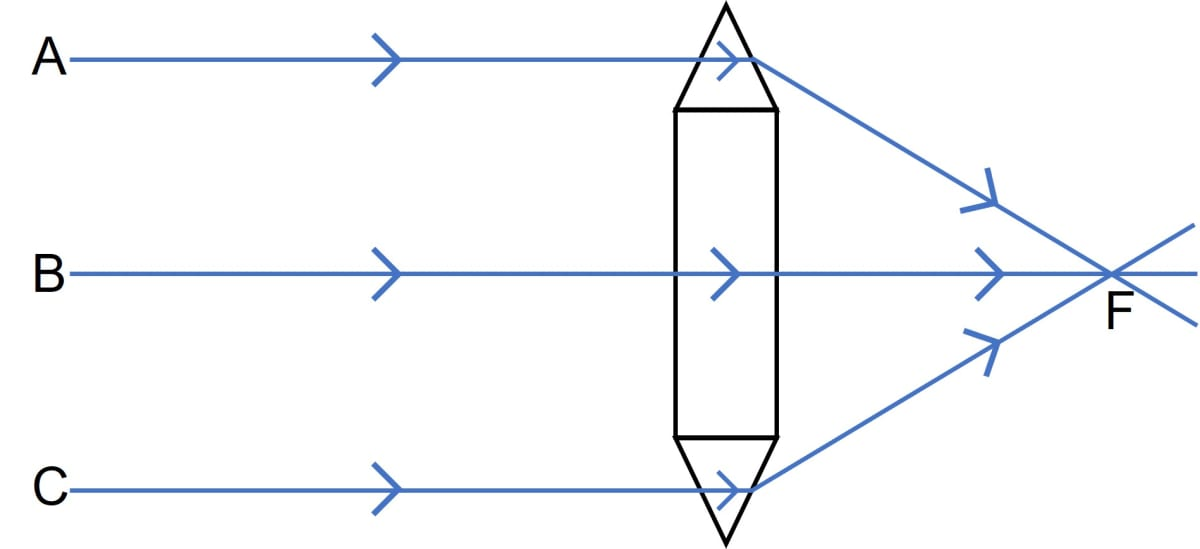#### Question 7

Show by a diagram the refraction of two light rays incident parallel to the principal axis on a concave lens by treating it as a combination of a glass slab and two triangular glass prisms.

Below diagram shows the refraction of two light rays incident parallel to the principal axis on a concave lens. The concave lens is shown as a combination of a glass slab and two triangular glass prisms: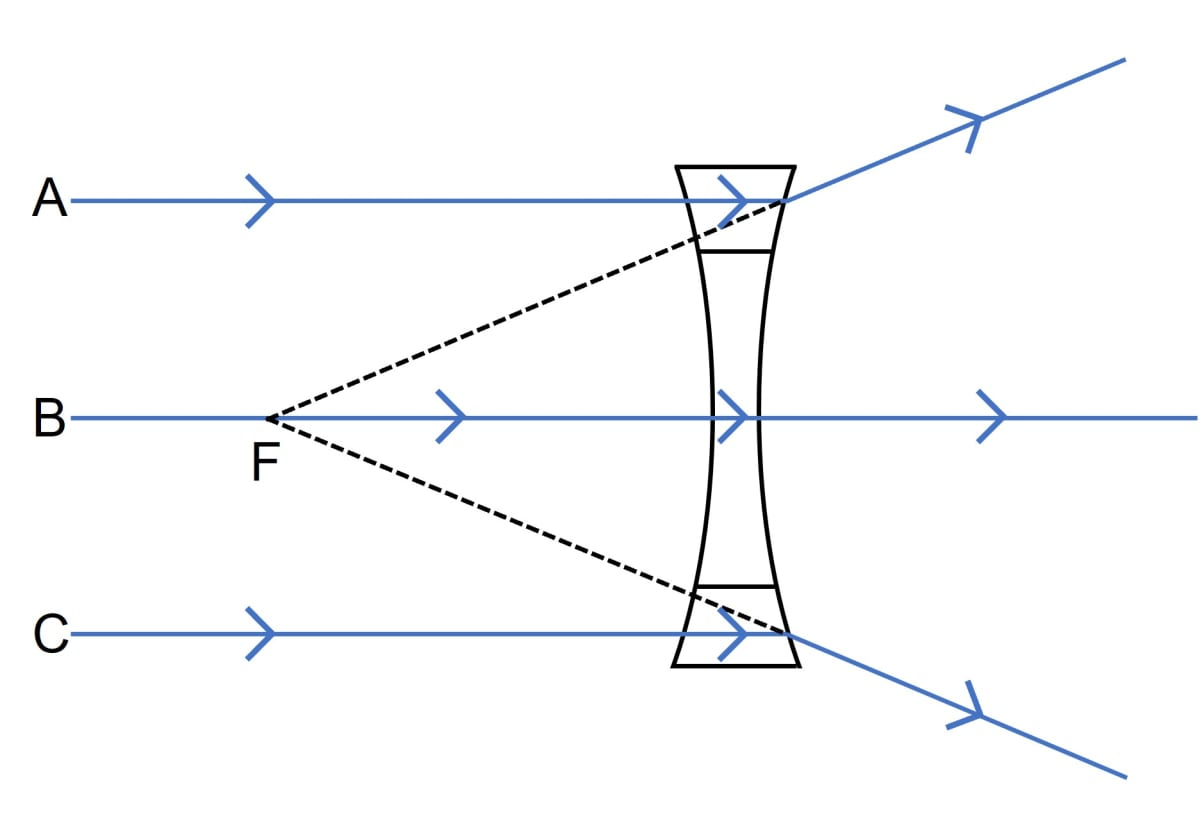#### Question 8

How does the action of the convex lens differ from that of a concave lens on a parallel beam of light incident on them? Draw diagrams to illustrate your answer.

Convex LensConcave Lens
In the case, when a parallel beam of light ray is incident on a convex lens, the prism in the upper part bends the incident ray downwards.In the case, when a parallel beam of light ray is incident on a concave lens, the upper part of the lens bends the incident ray upwards.
The ray incident on the lower part of the prism is bent in upward direction.The ray incident on the lower part of the prism is bent in downward direction
The central part which is a parallel-sided glass slab passes the ray undeviated.The central part which is a parallel-sided glass slab passes the ray undeviated.

Convergent action of Convex lens is shown in the below diagram: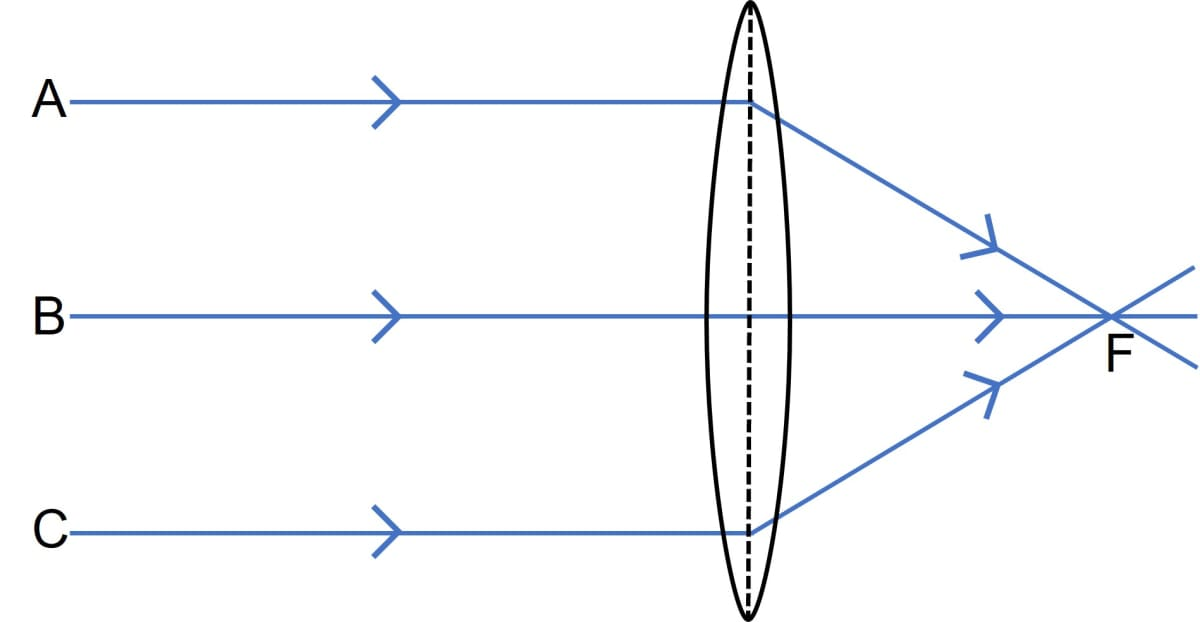Divergent action of Concave lens is shown in the below diagram:#### Question 9

Define the term principal axis of a lens.

The line joining the centres of curvature of the two surfaces of the lens is called the principal axis.

#### Question 10

Explain the optical centre of a lens with the help of proper diagram(s).

Optical centre is a point on the principal axis of the lens such that a ray of light passing through this point emerges parallel to its direction of incidence. The optical centre is thus the centre of the lens.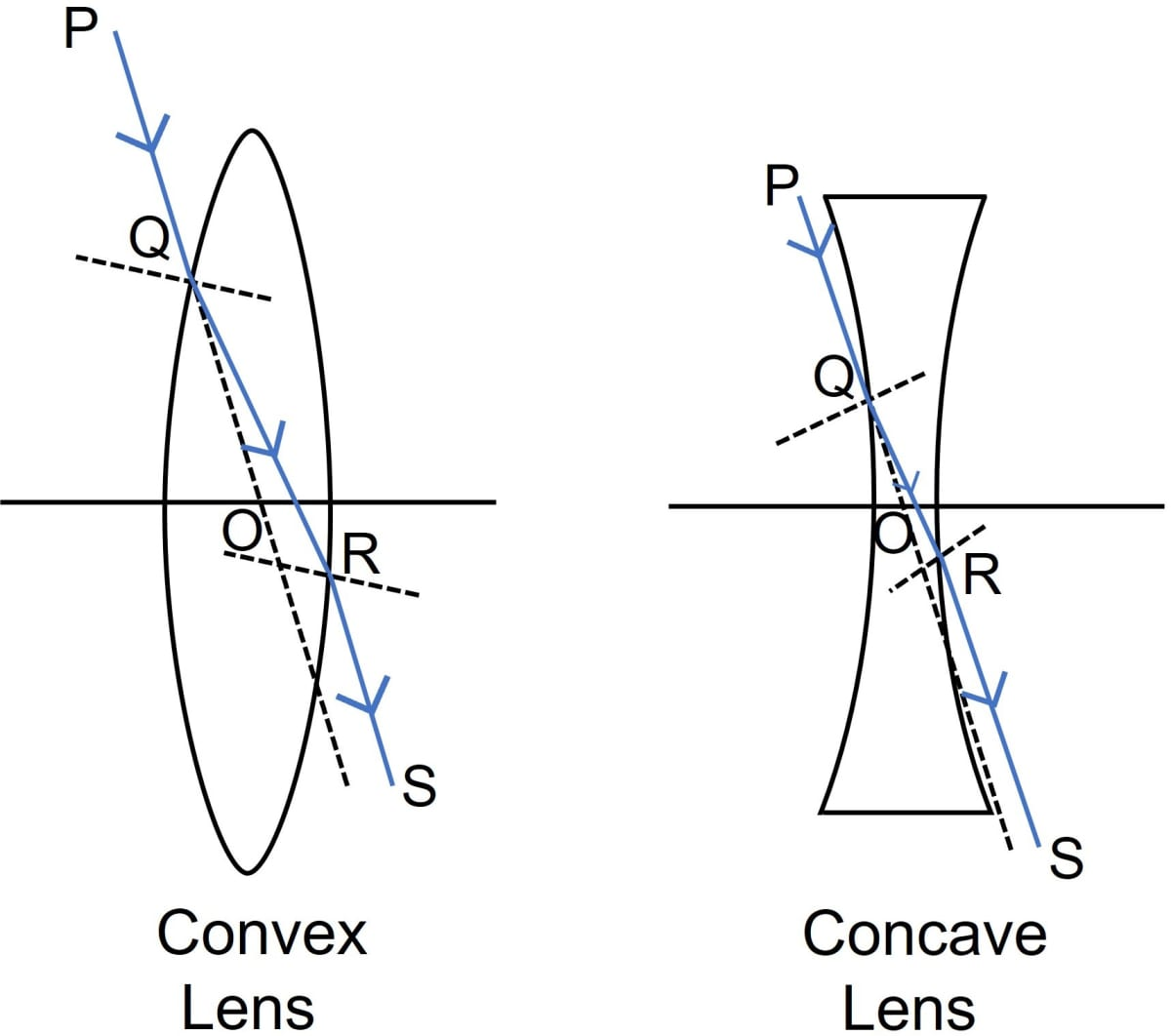The point O is the optical centre of the convex and concave lenses .

#### Question 11

A ray of light incident at a point on the principal axis of a convex lens passes undeviated through the lens.

(a) What special name is given to this point on the principal axis?

(b) Draw a labelled diagram to support the answer in part (a).

(a) This point on the principal axis is called Optical Centre.

(b) Below labelled diagram shows the Optical Centre for Convex and Concave lens: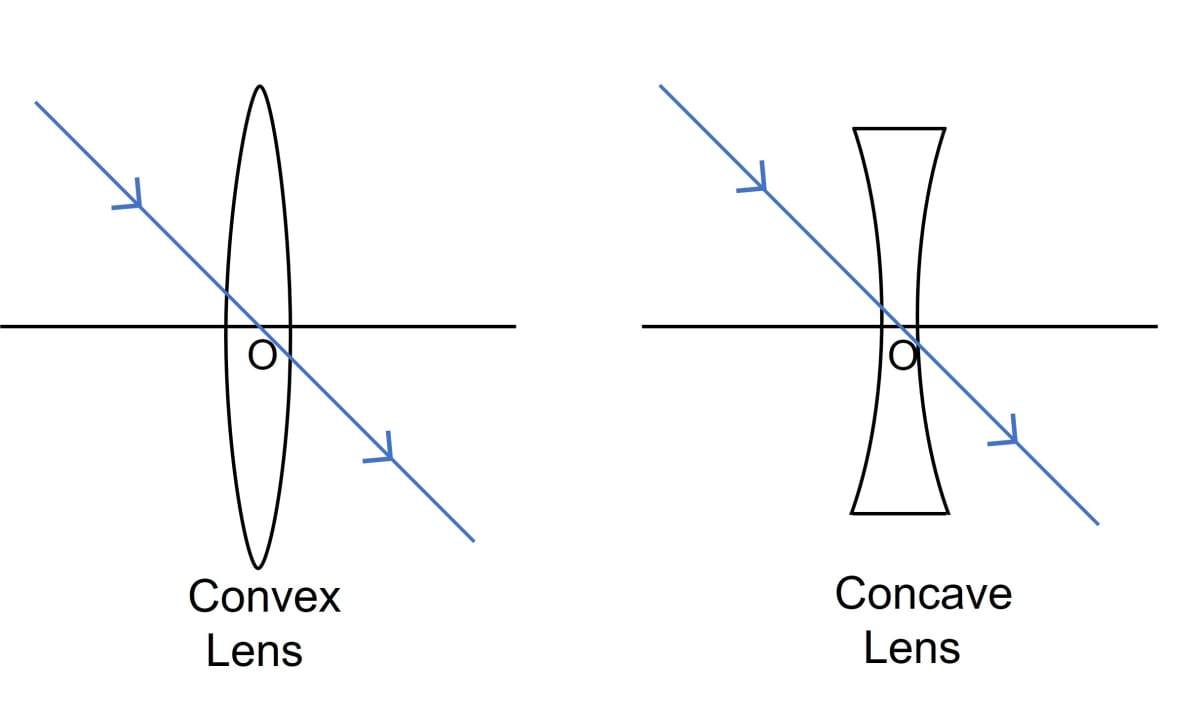#### Question 12

State the condition when a lens is called equiconvex or equi-concave.

A lens is called an equiconvex or equi-concave lens, when the radii of curvature of the two surfaces of lens are equal.

#### Question 13

Define the term principal foci of a convex lens and illustrate your answer with the aid of proper diagrams.

A light ray can enter a lens from either side, therefore, a lens has two principal foci, one on either side of the lens. These are known as first focal point( or first focus) F1 and the second focal point ( or second focus ) F2

For a convex lens, the first focal point is a point F1 on the principal axis of the lens such that the rays of light coming from it, after refraction through the lens, become parallel to the principal axis of the lens.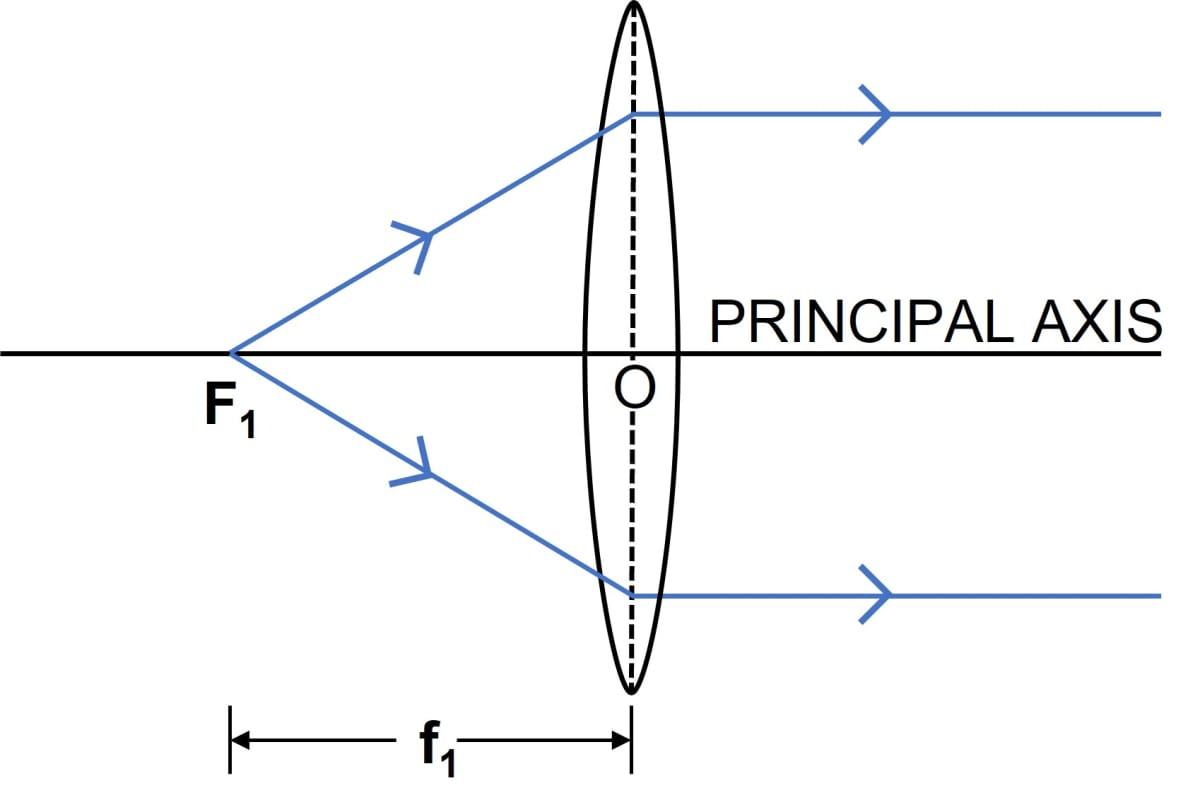For a convex lens, the second focal point is a point F2 on the principal axis of the lens such that the rays of light incident parallel to the principal axis, after refraction from the lens, pass through it.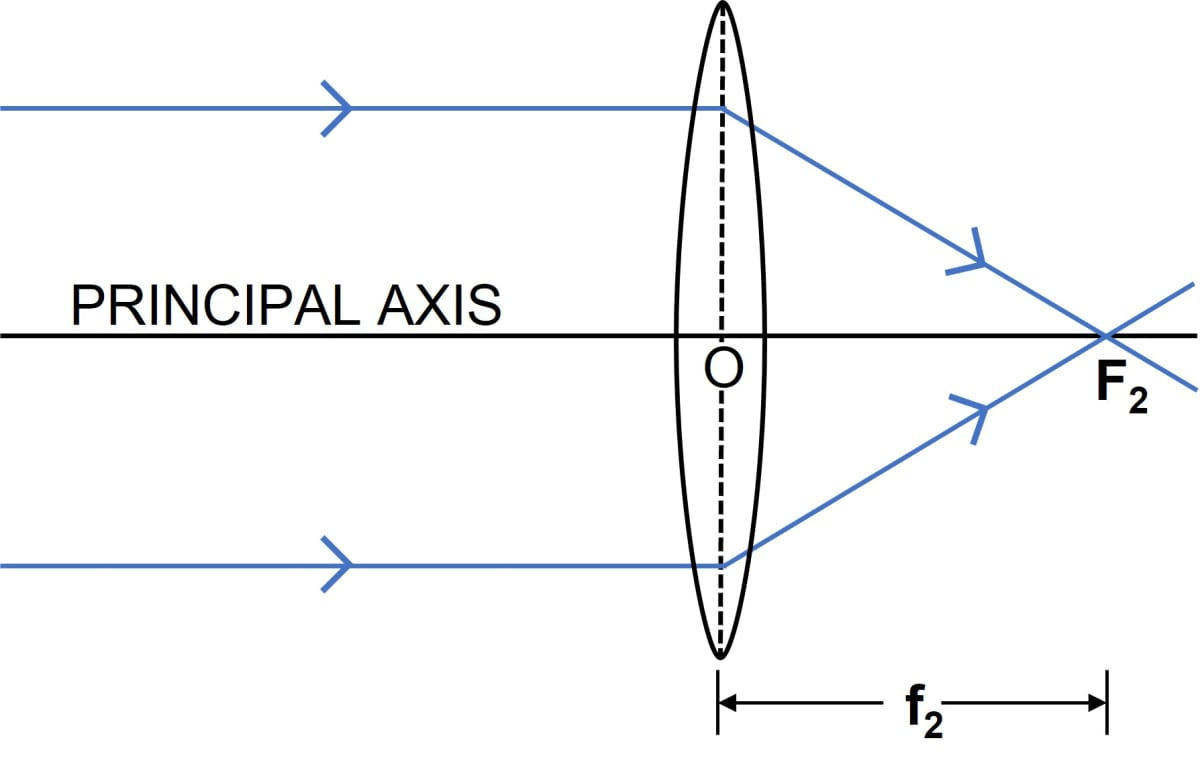#### Question 14

Define the term principal foci of a concave lens and show them with the help of proper diagrams.

A light ray can enter a lens from either side, therefore, a lens has two principal foci, one on either side of the lens. These are known as first focal point( or first focus) F1 and the second focal point ( or second focus ) F2

For a concave lens, the first focal point is a point F1 on the principal axis of a lens such that the incident rays of light appearing to meet at it, after refraction from the lens become parallel to the principal axis of the lens.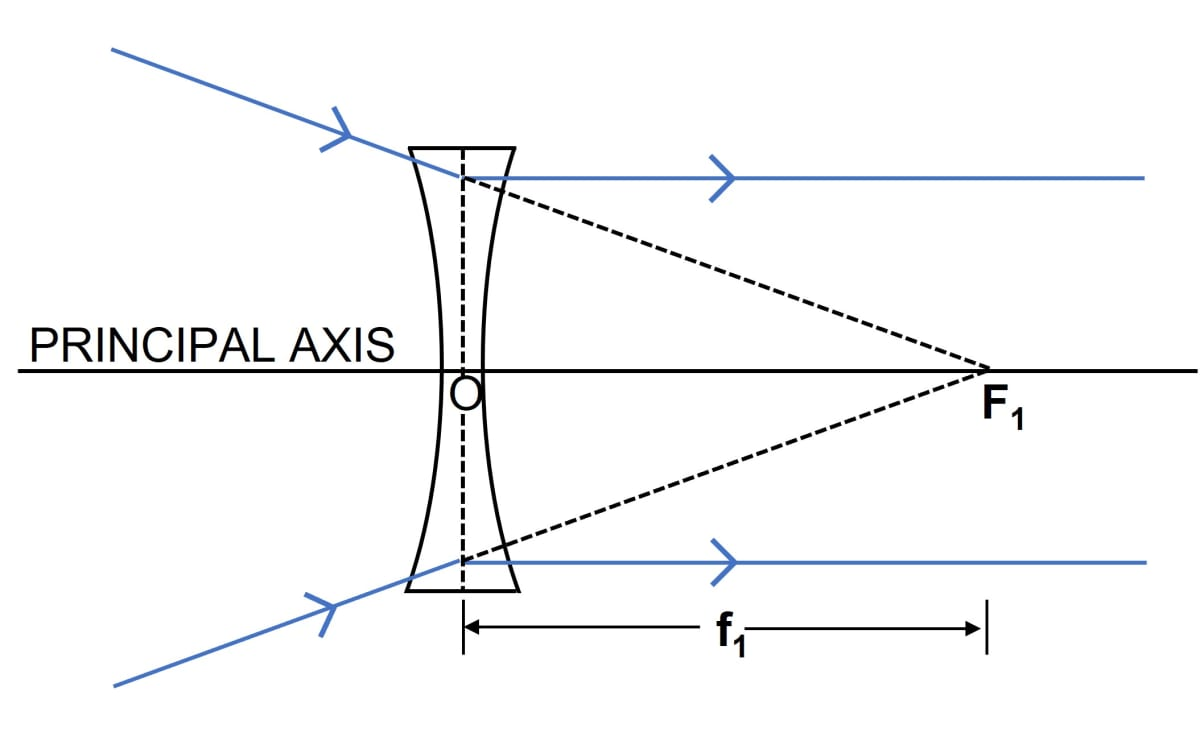For a concave lens, the second focal point is a point F2 on the principal axis of the lens such that the rays of light incident parallel to the principal axis, after refraction from the lens, appear to be diverging from this point.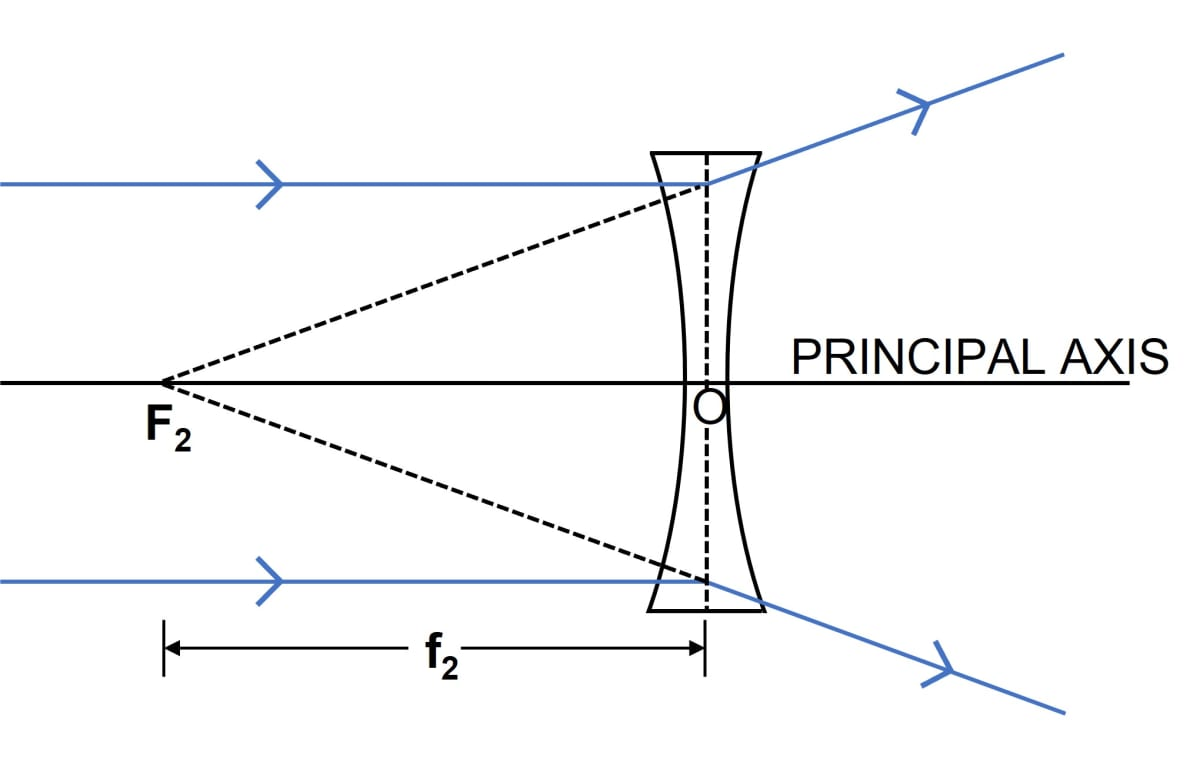#### Question 15

Draw a diagram to represent the second focus of a concave lens.

Below diagram represents the second focus of a concave lens:#### Question 16

Draw a diagram to represent the second focus of a convex lens.

Below diagram represents the second focus of a convex lens:#### Question 17

A ray of light, after refraction through a concave lens emerges parallel to the principal axis.

(a) Draw a ray diagram to show the incident ray and its corresponding emergent ray.

(b) The incident ray when produced meets the principal axis at a point F. Name the point F.

(a) Below diagram shows the incident ray and its corresponding emergent ray:(b) The incident ray when produced meets the principal axis at a point F, which is called as the first focus .

#### Question 18

A ray of light, after refraction through a convex lens emerges parallel to the principal axis.

(a) Draw a ray diagram to show it.

(b) The incident ray passes through a point F on the principal axis. Name the point F.

(a) Below ray diagram shows the incident ray and its corresponding emergent ray:(b) The point through which the incident ray passes is known as the first focus.

#### Question 19

A beam of light incident on a convex lens parallel to its principal axis converges at a point F on the principal axis. Name the point F. Draw a ray diagram to show it.

The point at which a beam of light incident on a convex lens parallel to its principal axis converges is called the second focus. Below ray diagram shows the second focus of a convex lens:#### Question 20

A beam of light incident on a thin concave lens parallel to its principal axis diverges and appears to come from a point F on the principal axis. Name the point F. Draw a ray diagram to show it.

The point at which a beam of light incident on a concave lens parallel to its principal axis converges is called the second focus. Below ray diagram shows the second focus of a concave lens:#### Question 21

Define the term focal length of a lens.

The distance of focus from the optical centre of the lens, is called its focal length.

A lens has two focal length.

1. First focal length — the distance from the optical centre O of the lens to its first focal point F1 is called the first focal length.
2. Second focal length — the distance from the optical centre O of the lens to its second focal point F2 is called the second focal length.

#### Question 22

What do you mean by focal plane of a lens?

A plane normal to the principal axis, passing through the focus, is called the focal plane of a lens.

A lens has two focal plane.

(a) A plane passing through the first focal point and normal to the principal axis of the lens is called the first focal plane.

(a) A plane passing through the second focal point and normal to the principal axis of the lens is called the second focal plane.

#### Question 23

State the condition for each of the following :

(a) a lens has both its focal lengths equal.

(b) a ray passes undeviated through the lens.

(a) When the medium is the same on either side of the lens then it has both its focal lengths equal.

(b) A ray passes undeviated through the lens, when it is incident at the optical centre of the lens.

#### Question 24

A parallel oblique beam of light falls on a

(i) convex lens,

(ii) concave lens.

Draw a diagram in each case to show the refraction of light through the lens.

(i) Below diagram shows the refraction of a parallel oblique beam of light by a convex lens: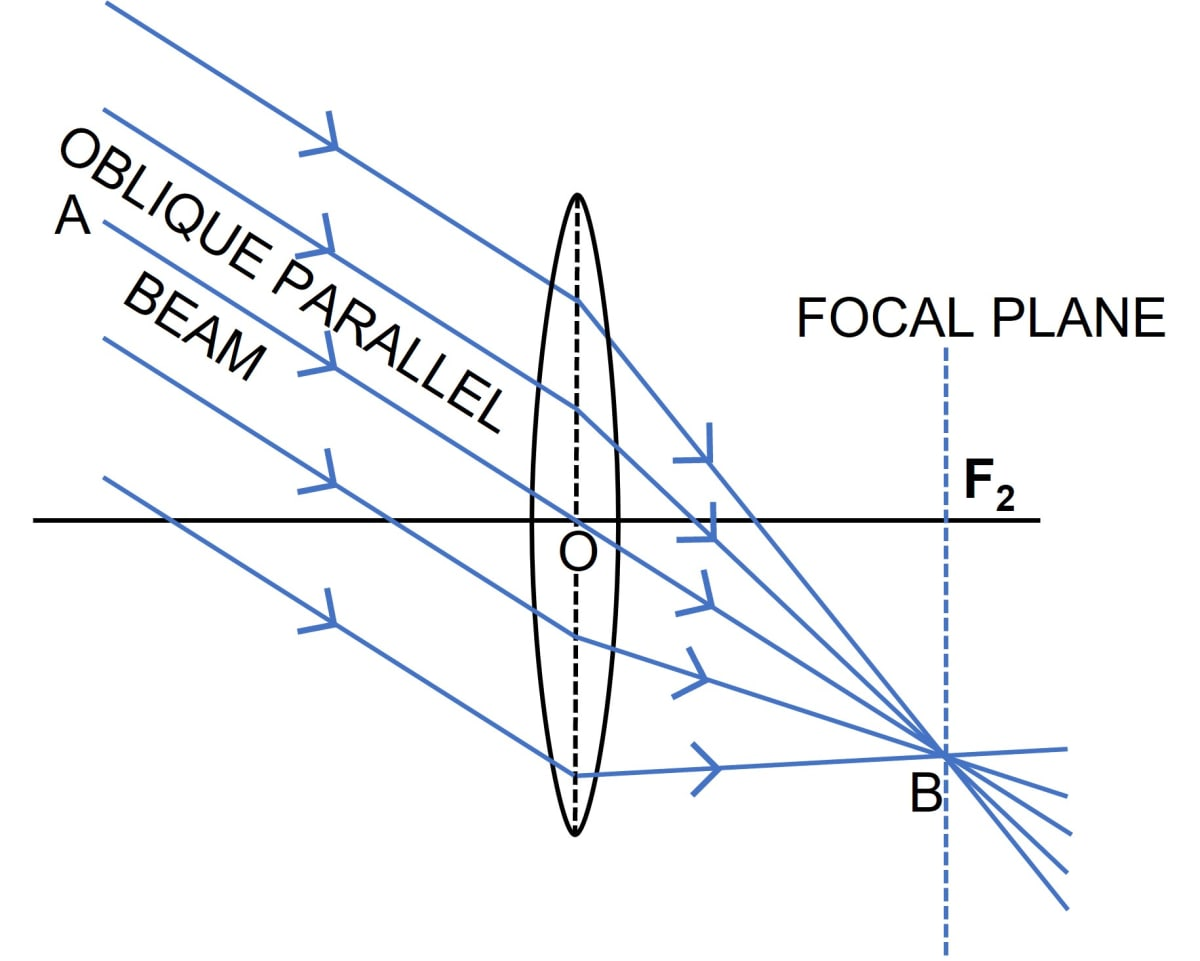(ii) Below diagram shows the refraction of a parallel oblique beam of light by a concave lens: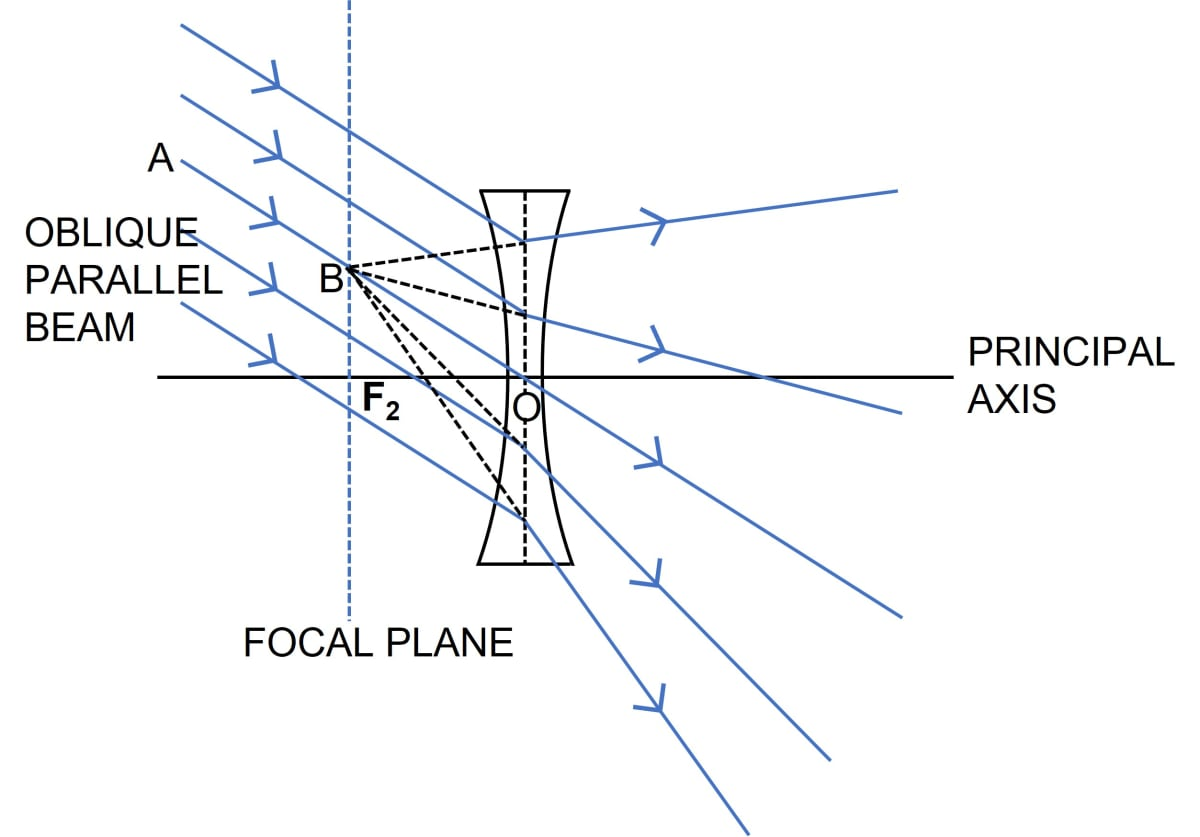#### Question 25

The diagram below shows a lens as a combination of a glass slab and two prisms.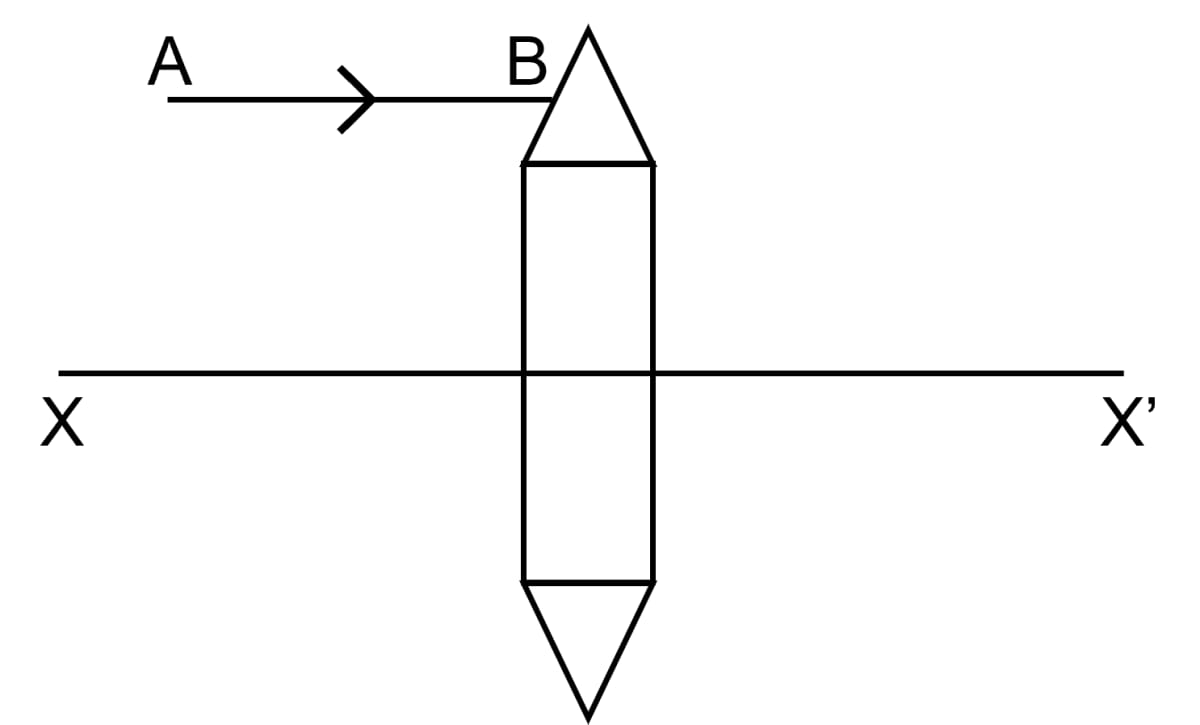(i) Name the lens formed by the combination.

(ii) What is the line XX' called?

(iii) Complete the ray diagram and show the path of the incident ray AB after passing through the lens.

(iv) The final emergent ray will either meet XX' at a point or appear to come from a point on XX'. Label the point as F. What is this point called?

(i) The lens formed by the combination is a convex lens.

(ii) The line XX' is called the principal axis.

(iii) Below diagram shows the path of the incident ray AB after passing through the convex lens: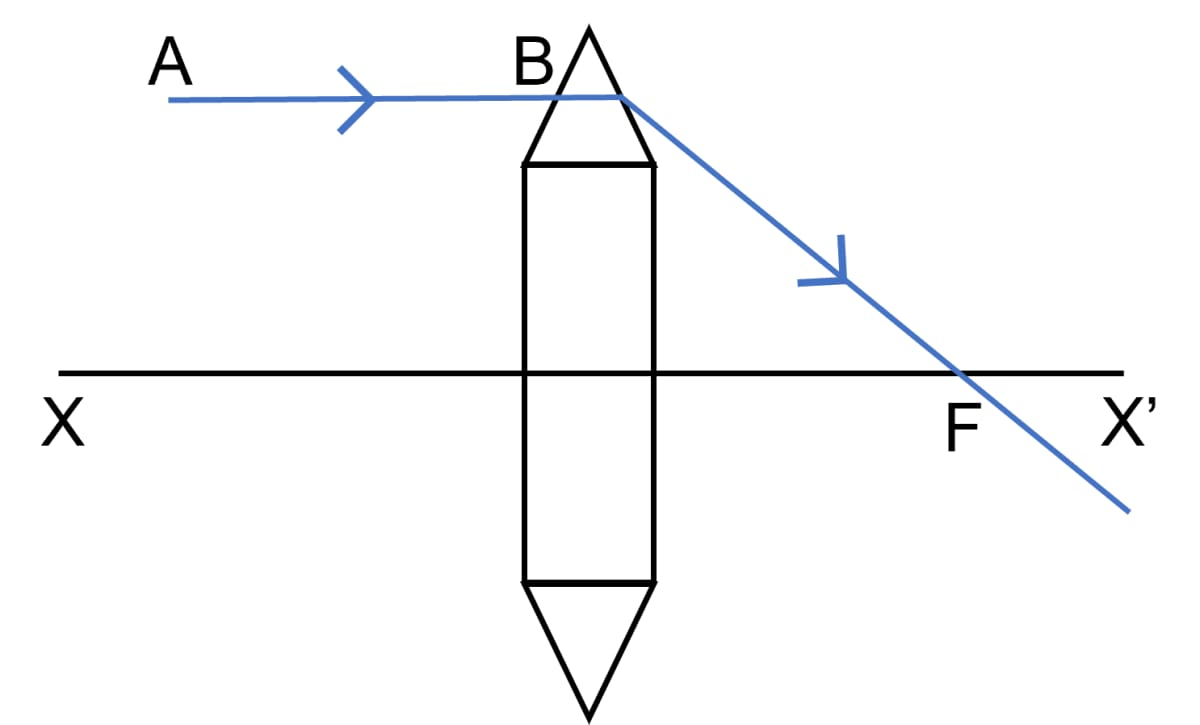(iv) The point F is called a focal point.

#### Question 26

The diagram below shows a lens as a combination of a glass slab and two prisms.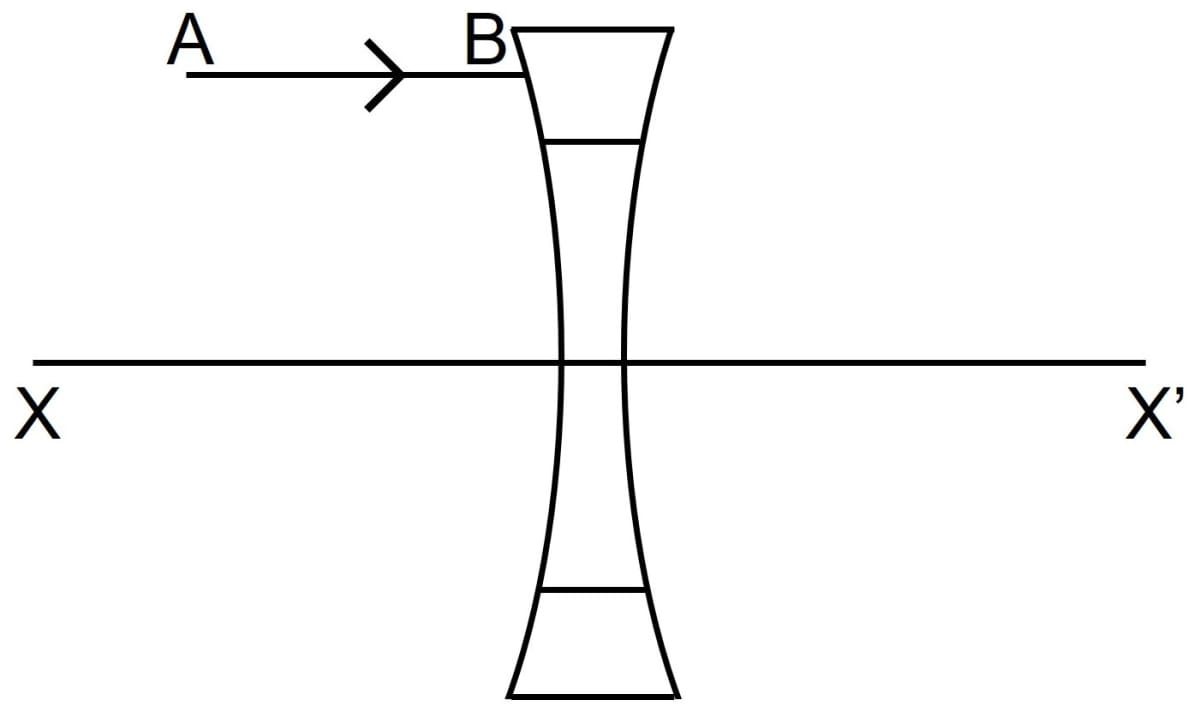(i) Name the lens formed by the combination.

(ii) What is the line XX' called?

(iii) Complete the path of the incident ray AB after passing through the lens.

(iv) The final emergent ray either meets XX' at a point or appears to come from a point on XX’. Label it as F. What is this point called?

(i) The combination forms a concave lens.

(ii) The line XX' is called the principal axis.

(iii) Below diagram shows the path of the incident ray AB after passing through the concave lens: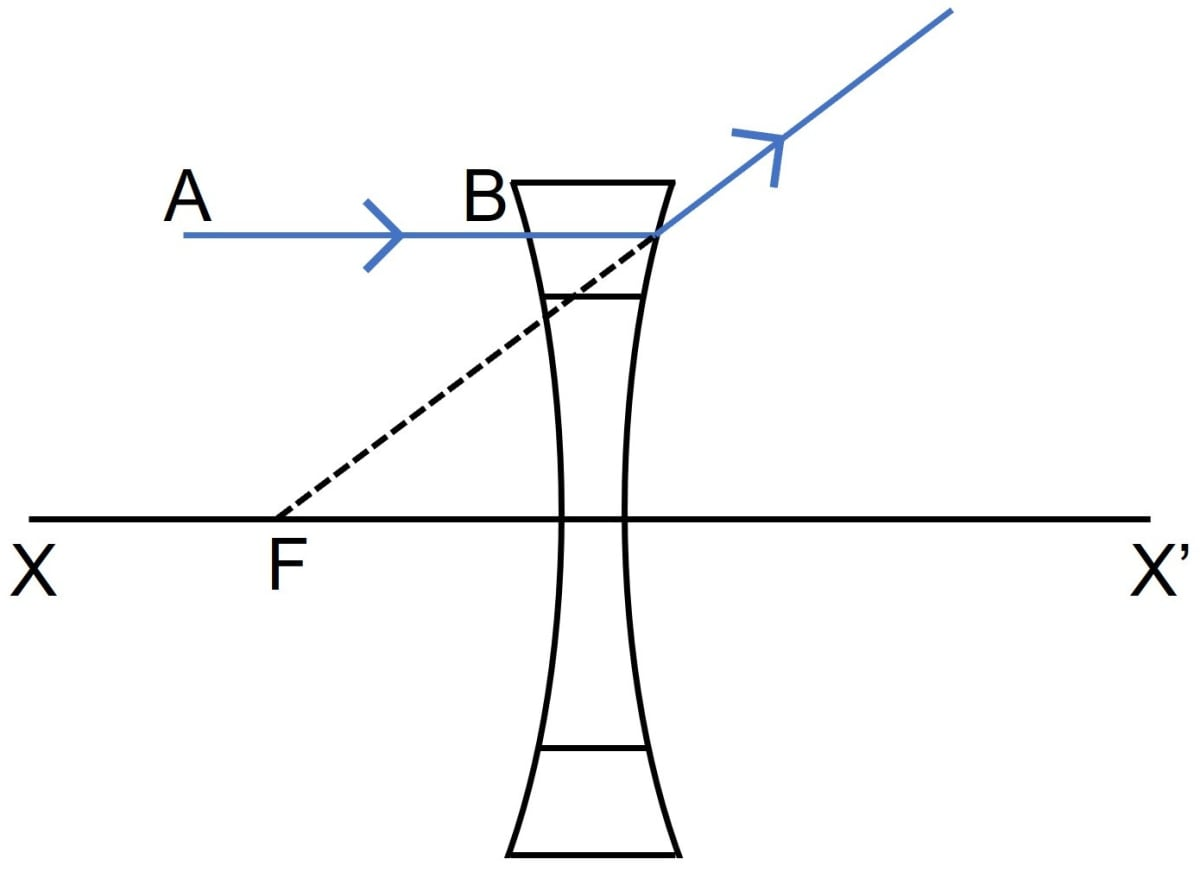(iv) The point F is called a focal point or focus.

#### Question 27

In figure (a) and (b), F1 and F2 are the positions of the two foci of thin lenses. Draw the path taken by the light ray AB after it emerges from each lens.

(a) Figure a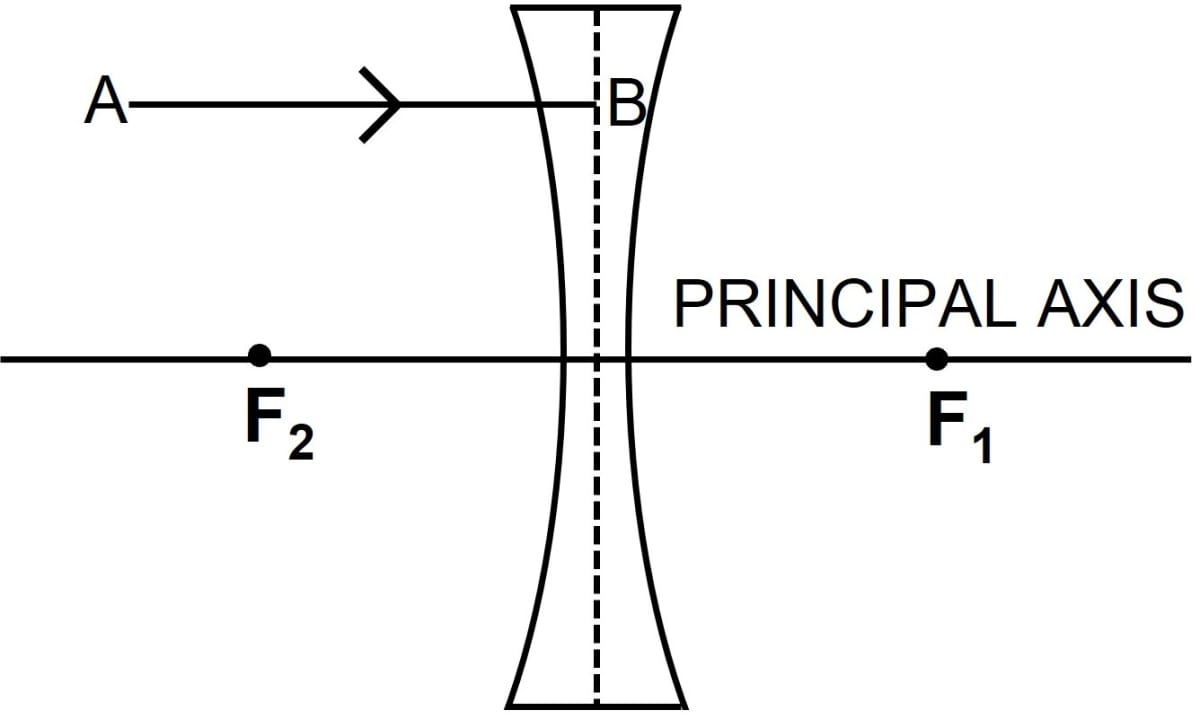(b) Figure b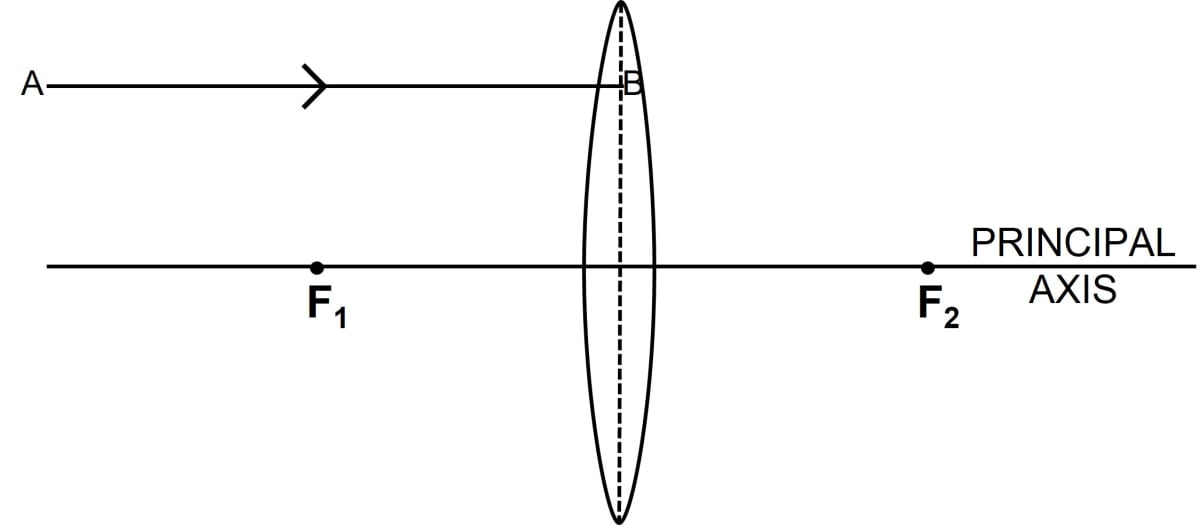(a) Below ray diagram shows the path taken by the light ray AB after it emerges from the thin concave lens: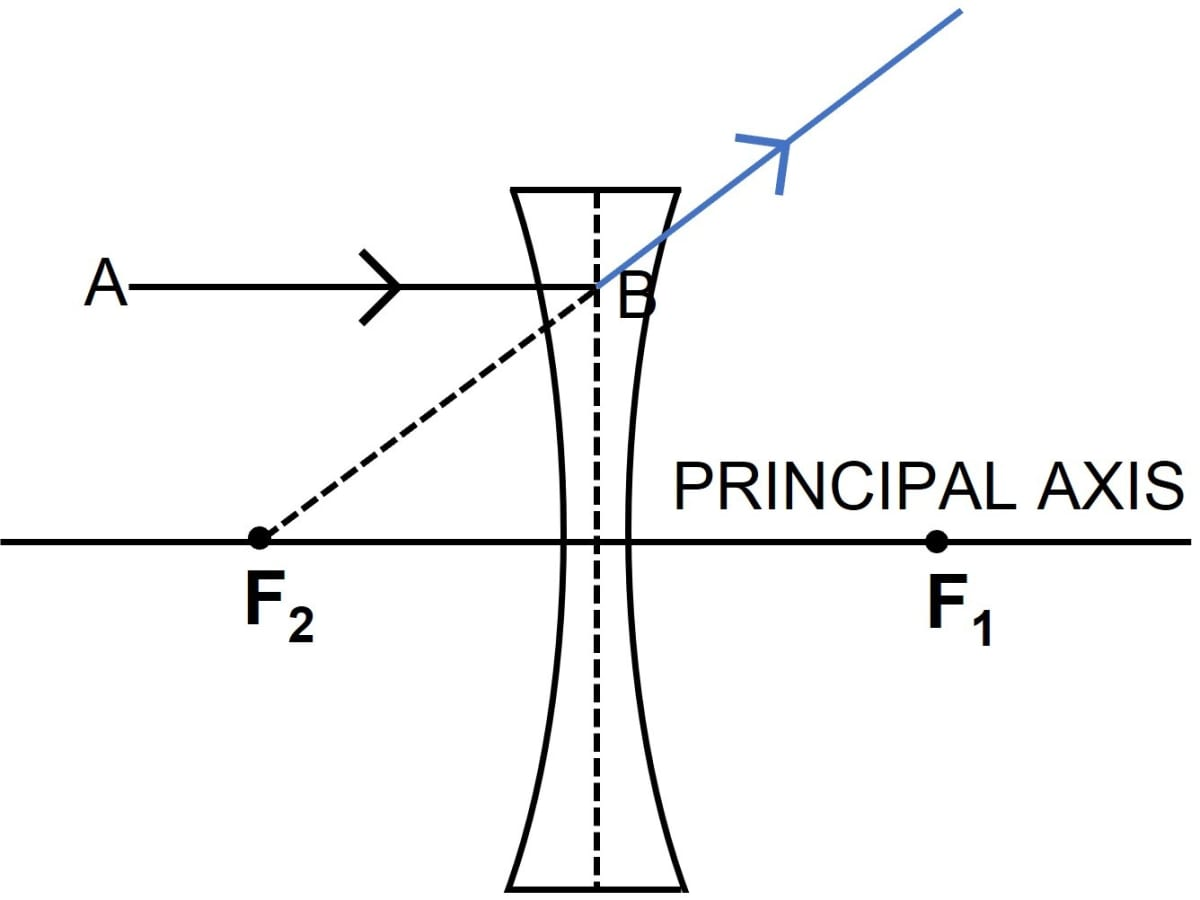(b) Below ray diagram shows the path taken by the light ray AB after it emerges from the thin convex lens: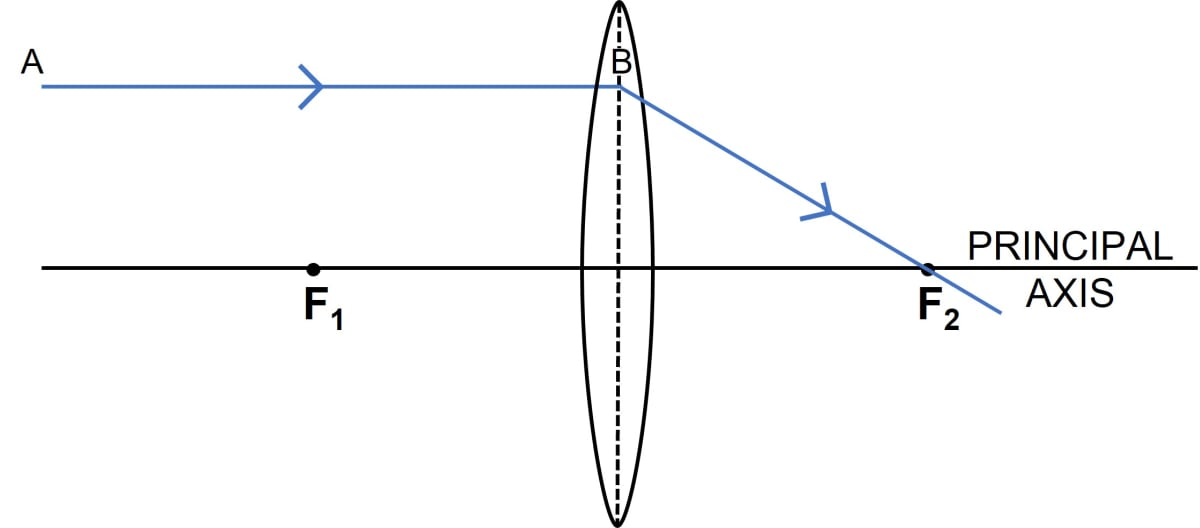#### Question 28

In figure (a) and (b), F1 and F2 are the two foci of thin lenses and AB is the incident ray. Complete the diagram to show the path of the ray AB after refraction through the lens.

(a) Figure a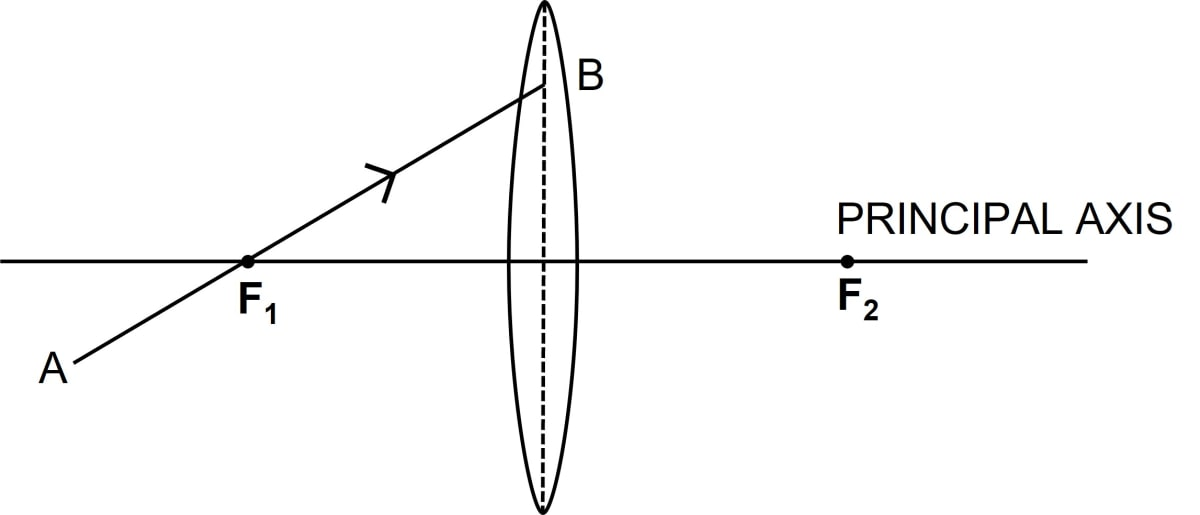(b) Figure b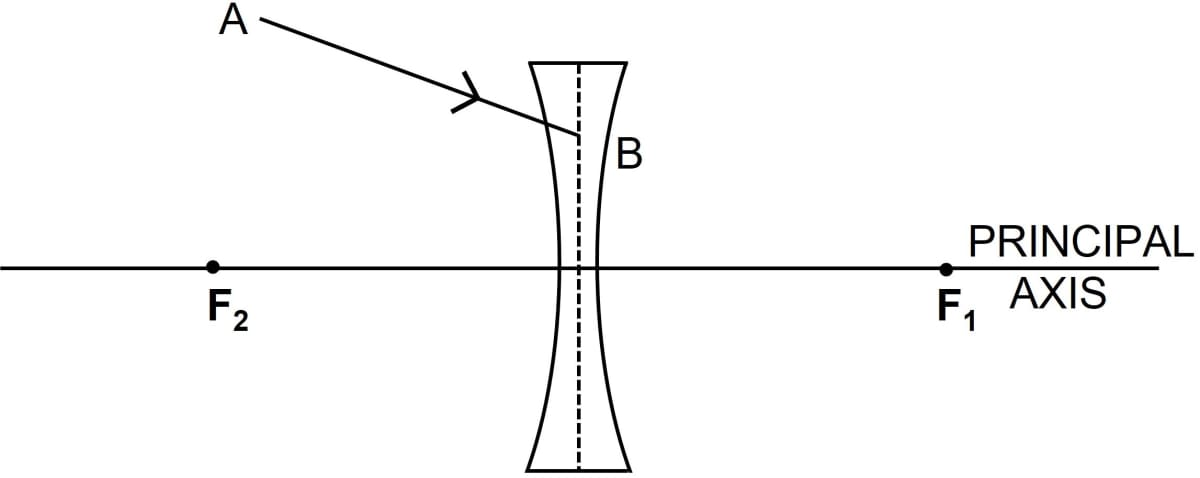(a) Below ray diagram shows the path taken by the light ray AB after refraction through the thin convex lens: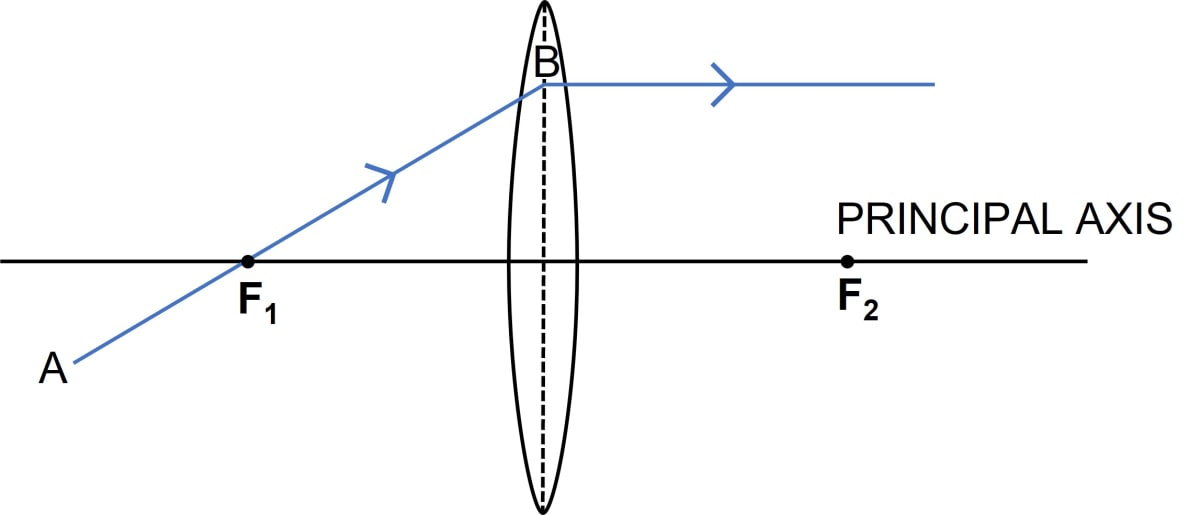(b) Below ray diagram shows the path taken by the light ray AB after refraction through the thin concave lens: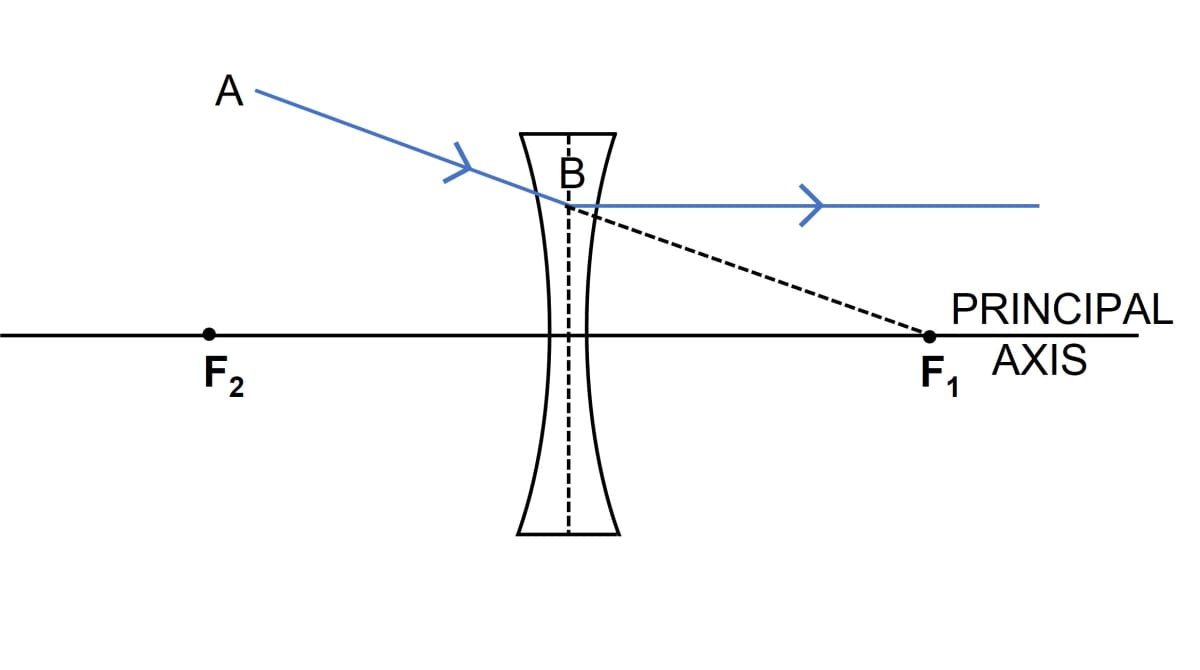#### Question 29

Complete the following sentences —

(a) If half part of a convex lens is covered, the focal length __________ change, but the intensity of image _________.

(b) A convex lens is placed in water. Its focal length will ________.

(c) The focal length of a thin convex lens is _________ than that of a thick convex lens.

(a) If half part of a convex lens is covered, the focal length does not change, but the intensity of the image decreases.

(b) A convex lens is placed in water. Its focal length will increase.

(c) The focal length of a thin convex lens is more than that of a thick convex lens.

## Multiple Choice Type

#### Question 1

A ray of light after refraction through a lens emerges parallel to the principal axis of the lens. The incident ray either passes through :

1. its optical centre
2. its first focus ✓
3. its second focus
4. the centre of curvature of the first surface

When the incident ray passes through the first focus then it emerges parallel to the principal axis of the lens after refraction. We can understand this better with the help of diagrams given below —

Convex LensConcave Lens#### Question 2

A ray of light incident on a lens parallel to its principal axis, after refraction passes through or appears to come from:

1. its first focus
2. its optical entre
3. its second focus ✓
4. the centre of curvature of its second surface.

A ray of light incident on a lens parallel to its principal axis, after refraction passes through or appears to come from its second focus. We can understand this better with the help of the diagrams given below —

Convex LensConcave Lens## Exercise 5(B)

#### Question 1

What are the three principal rays that are drawn to construct the ray diagram for the image formed by a lens? Draw diagrams to support your answer.

(i) A ray of light incident at the optical centre O of the lens passes undeviated through the lens.

Convex Lens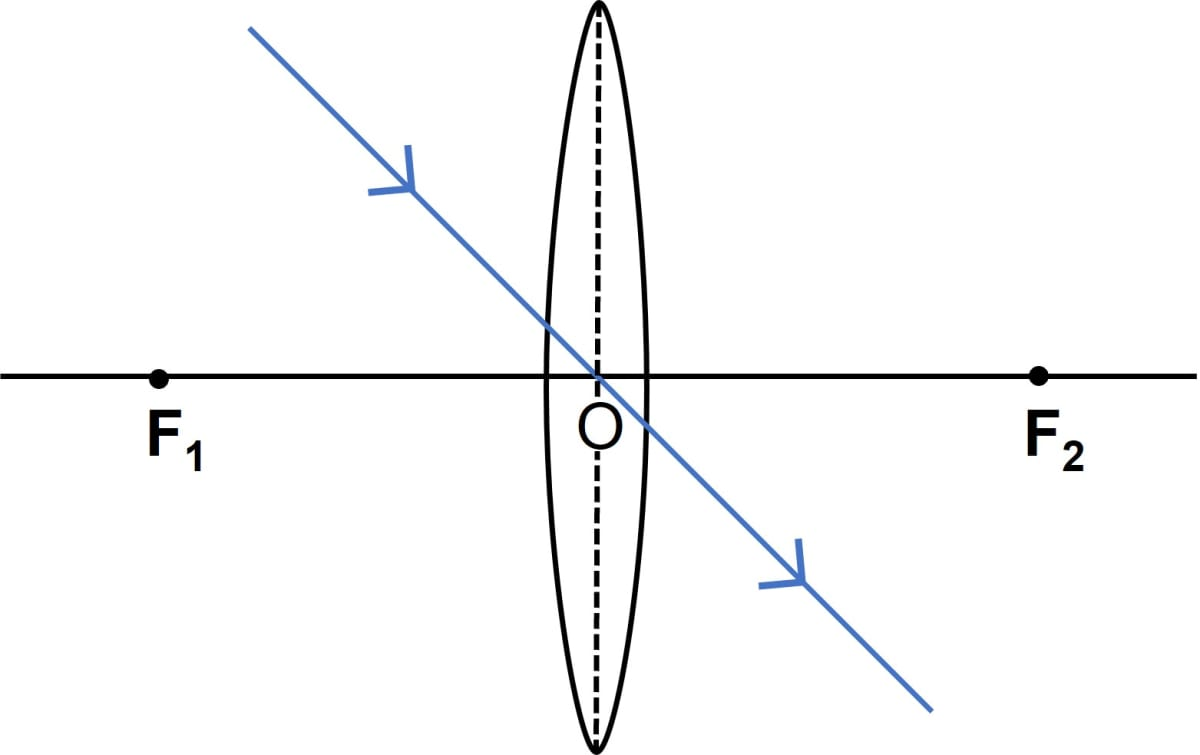Concave Lens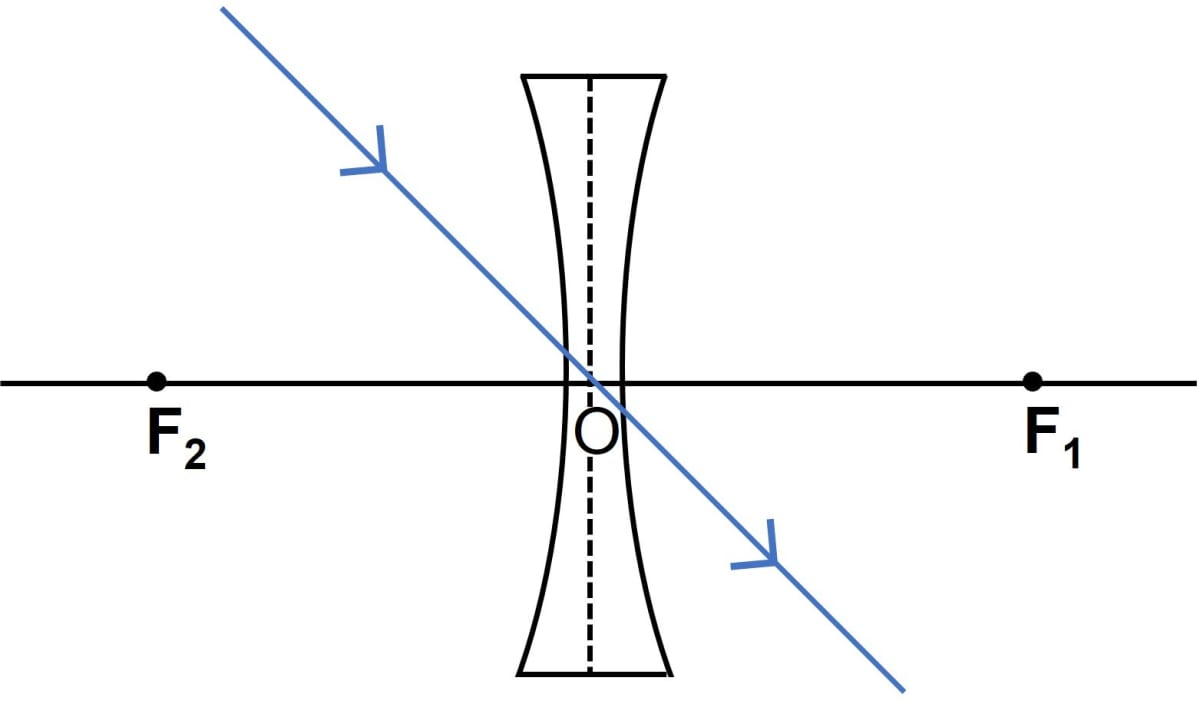(ii) A ray of light incident parallel to the principal axis of the lens, after refraction passes through the second focus F2 (in a convex lens) or appears to come from the second focus F2 (in a concave lens), respectively.

Convex Lens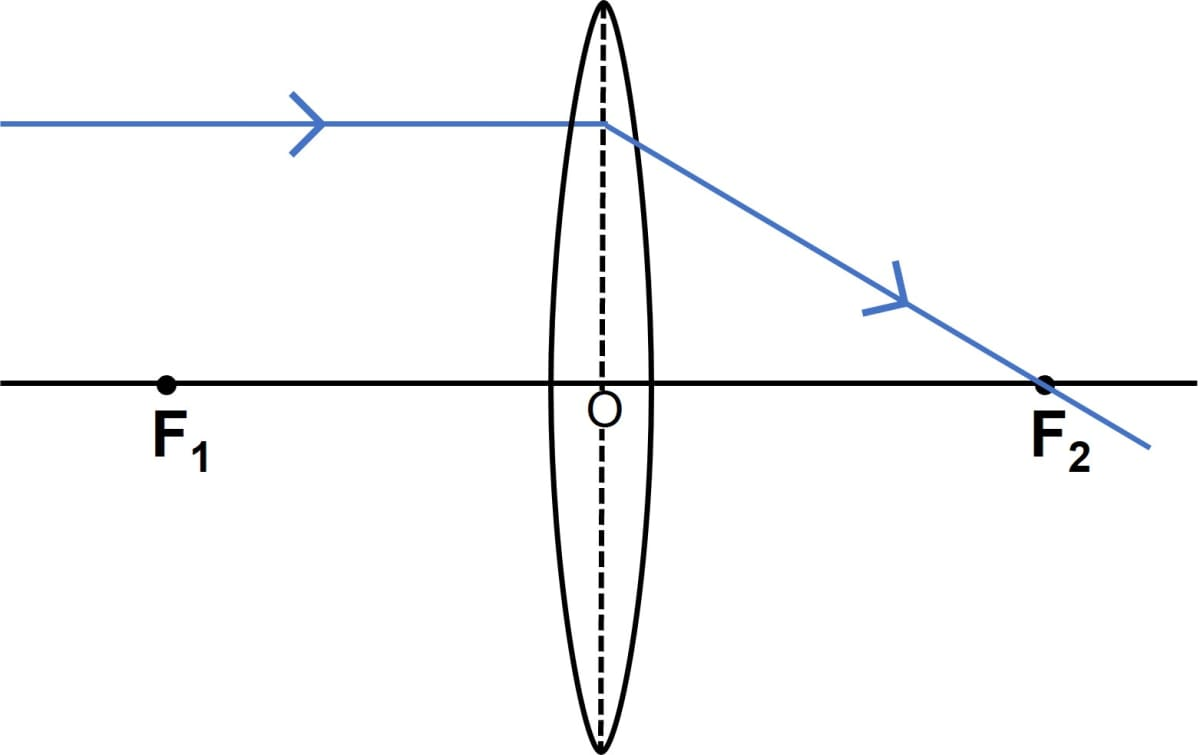Concave Lens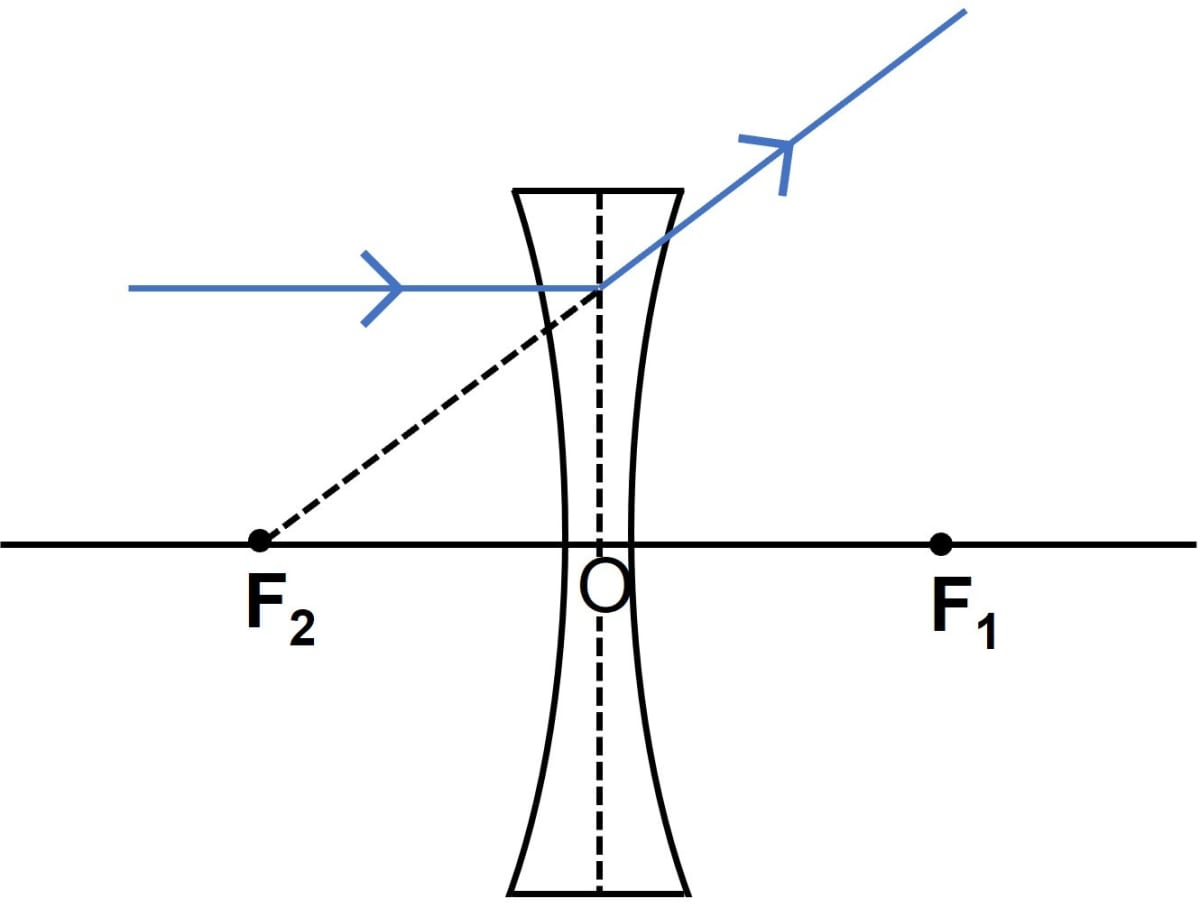(iii) A ray of light passing through the first focus F1 (in a convex lens) or directed towards the first focus F1 (in a concave lens), emerges parallel to the principal axis after refraction, respectively.

Convex Lens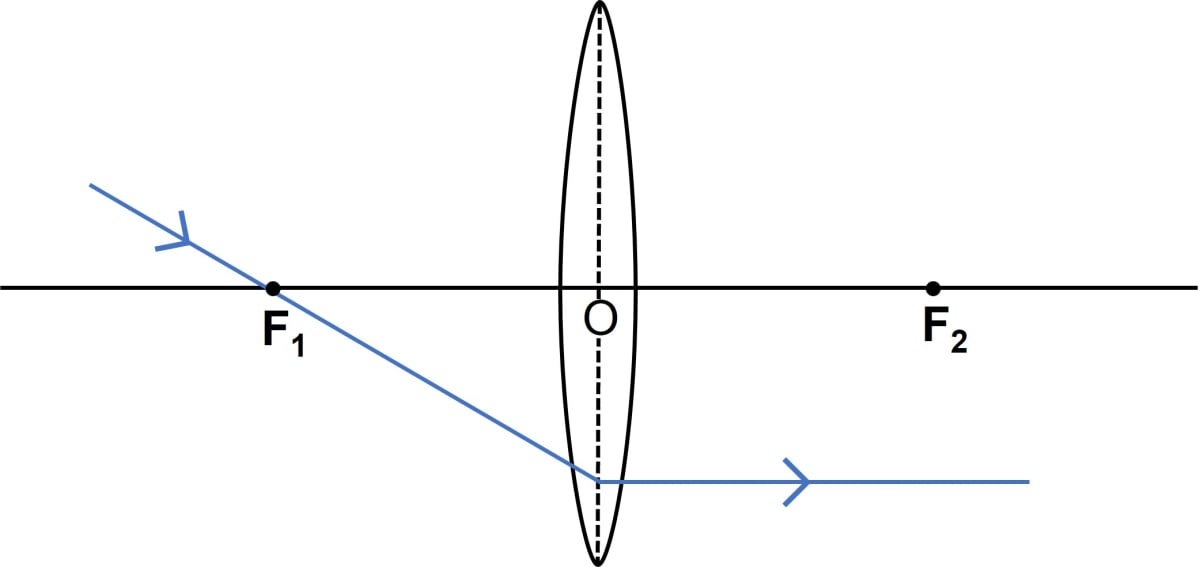Concave Lens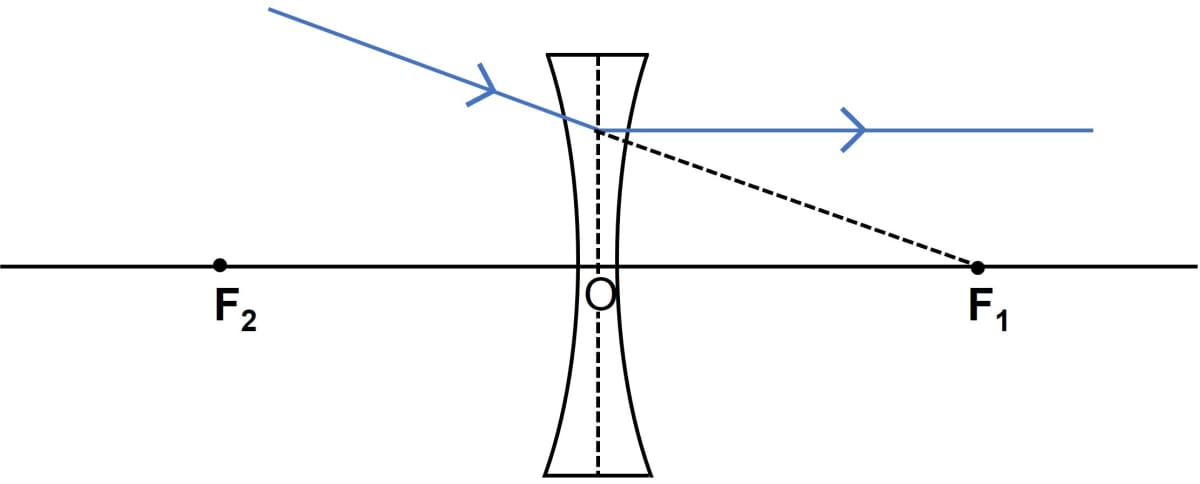#### Question 2

In the diagrams below, XX' represents the principal axis, O the optical centre and F the focus of the lens. Complete the path of the rays A and B as they emerge out of the lens.

(a)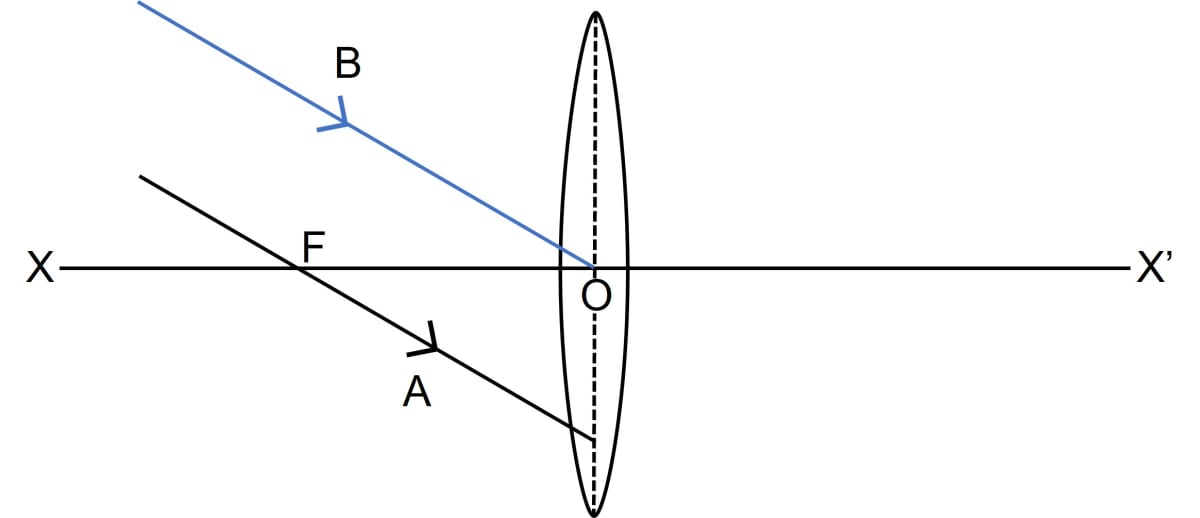(b)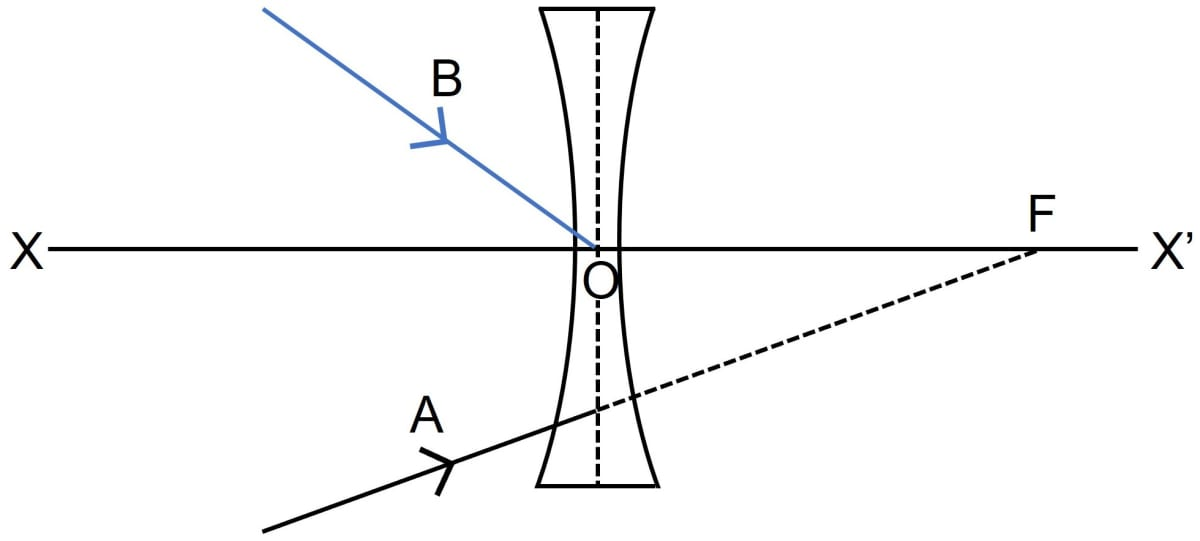Completed diagrams showing the path of the rays A and B as they emerge out of the lens are given below:

Convex Lens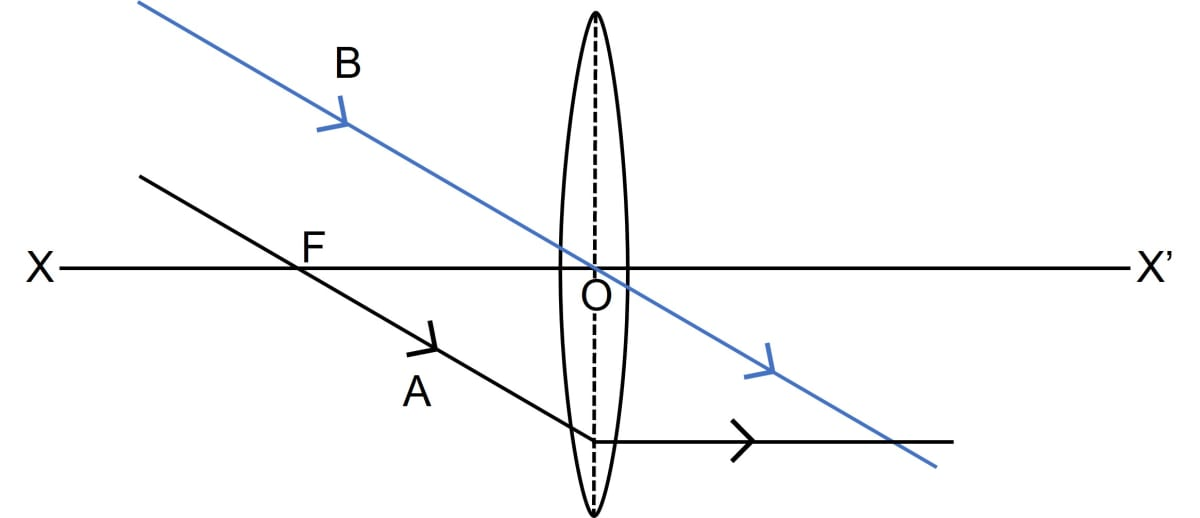Concave Lens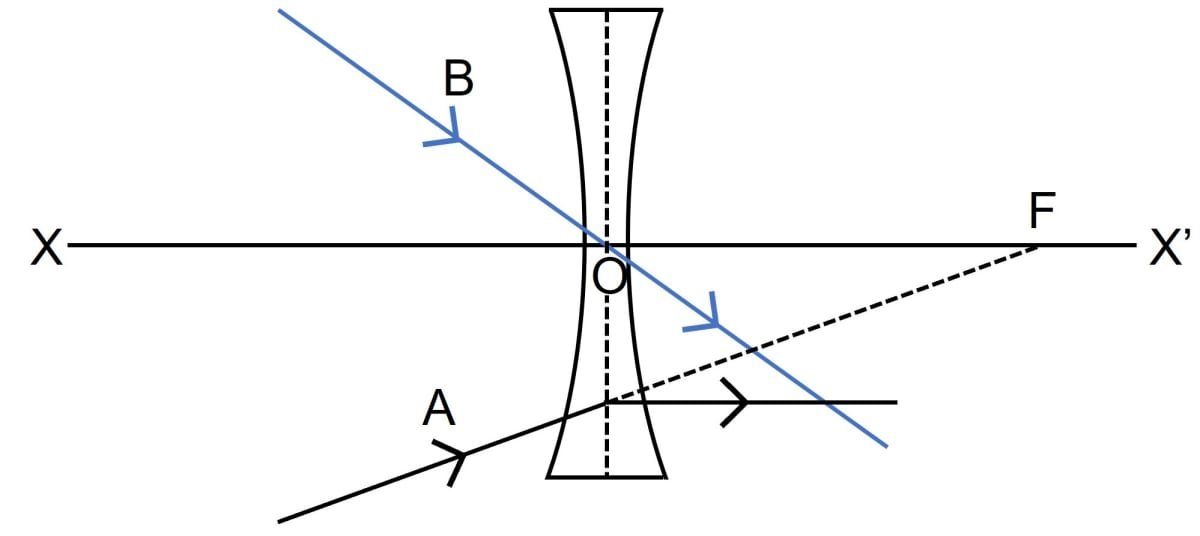#### Question 3

Where must a point source of light be placed in front of a convex lens so as to obtain a parallel beam of light?

In order to obtain a parallel beam of light, the source of light should be placed at the first focal point i.e. the focal point on the left of the optical centre of the convex lens.

#### Question 4

Distinguish between a real and a virtual image.

Real ImageVirtual Image
A real image is formed due to actual interaction of the rays refracted by the lens.A virtual image is formed when the rays refracted by the lens appear to move if they are produced backwards.
A real image can be obtained on a screen.A virtual image cannot be obtained on a screen.
A real image is inverted with respect to the object.A virtual image is erect with respect to the object.

#### Question 5

Study the diagram given below.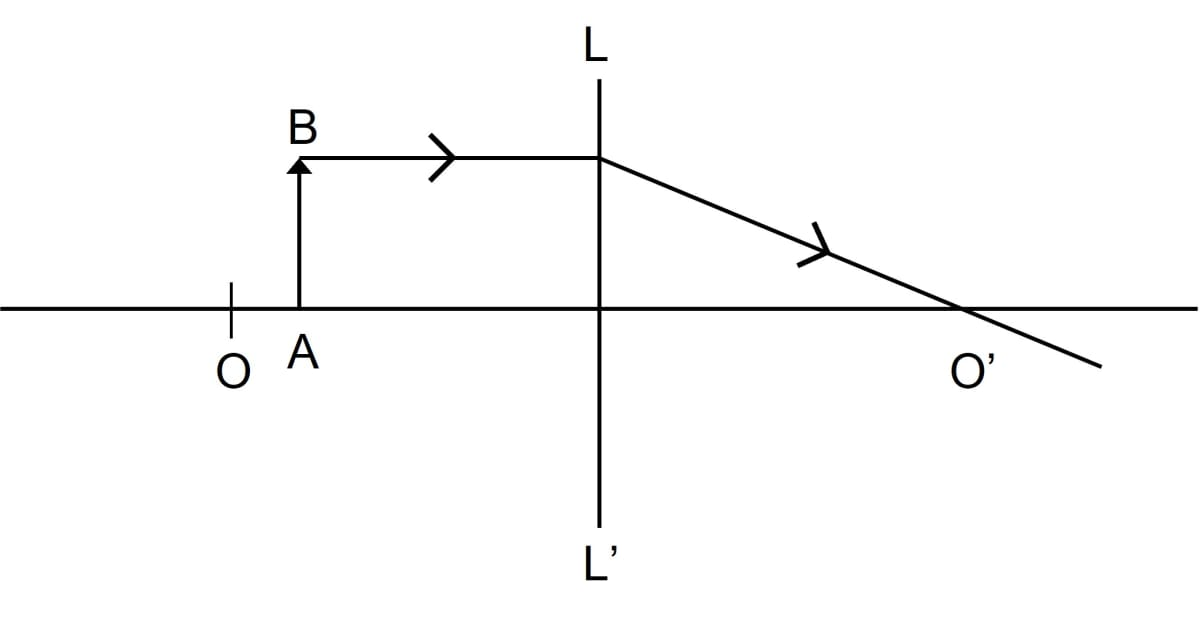(a) Name the lens LL'.

(b) What are the points O and O' called?

(c) Complete the diagram to form the image of the object AB.

(d) State the three characteristics of the image.

(e) Name a device in which this action of lens is used.

(a) LL' is a convex lens.

(b) The points O and O’ are called as first and second focal points.

(c) Completed diagram showing the formation of the image of the object AB is given below: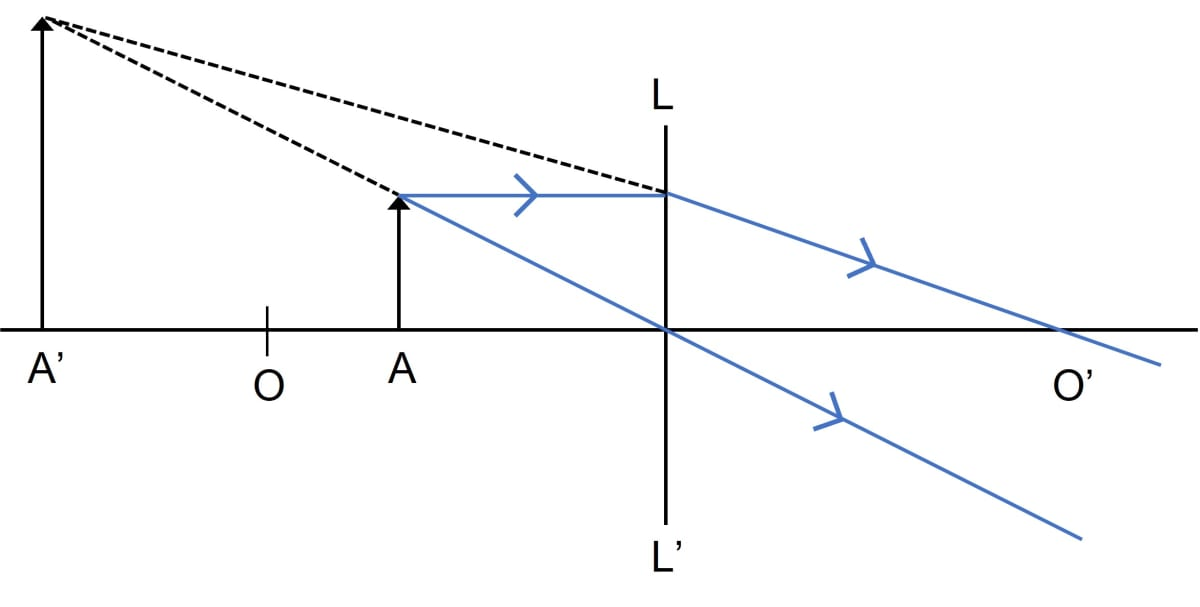(d) The three characteristics of the image are magnified, virtual and upright.

(e) This action of lens is used in the magnifying glass.

#### Question 6

Study the diagram below.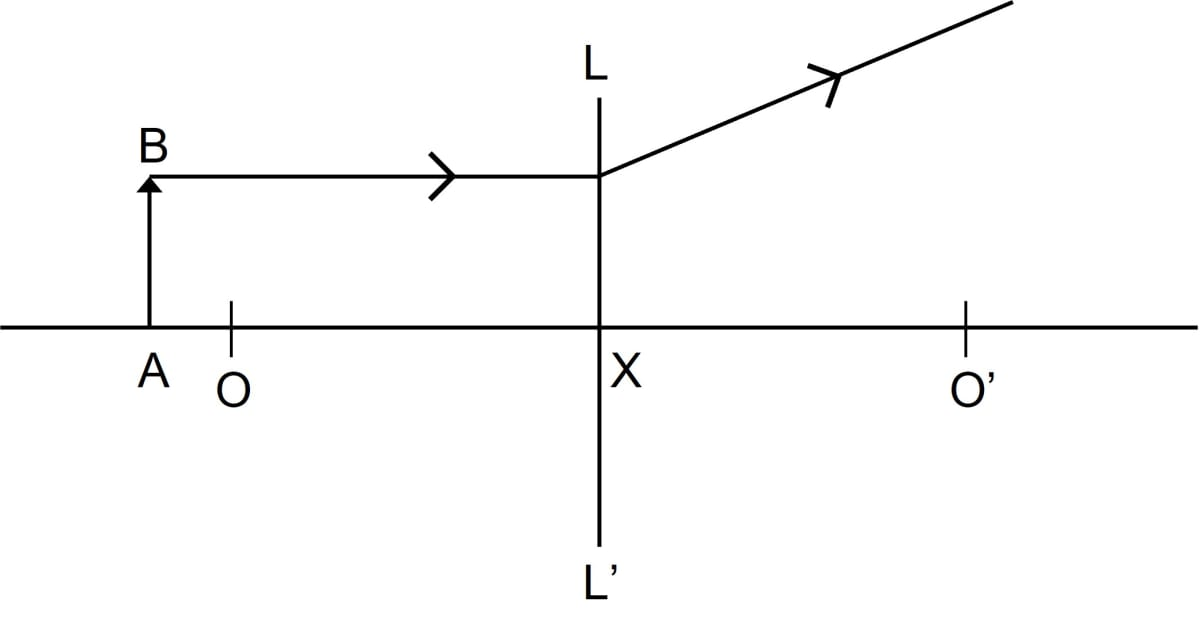(i) Name the lens LL'.

(ii) What are the points O and O' called?

(iii) Complete the diagram to form the image of the object AB.

(iv) State three characteristics of the image.

(i) LL' is a concave lens.

(ii) The points O and O' are called the second and first focal point.

(iii) Below is the completed diagram showing the image of the object AB: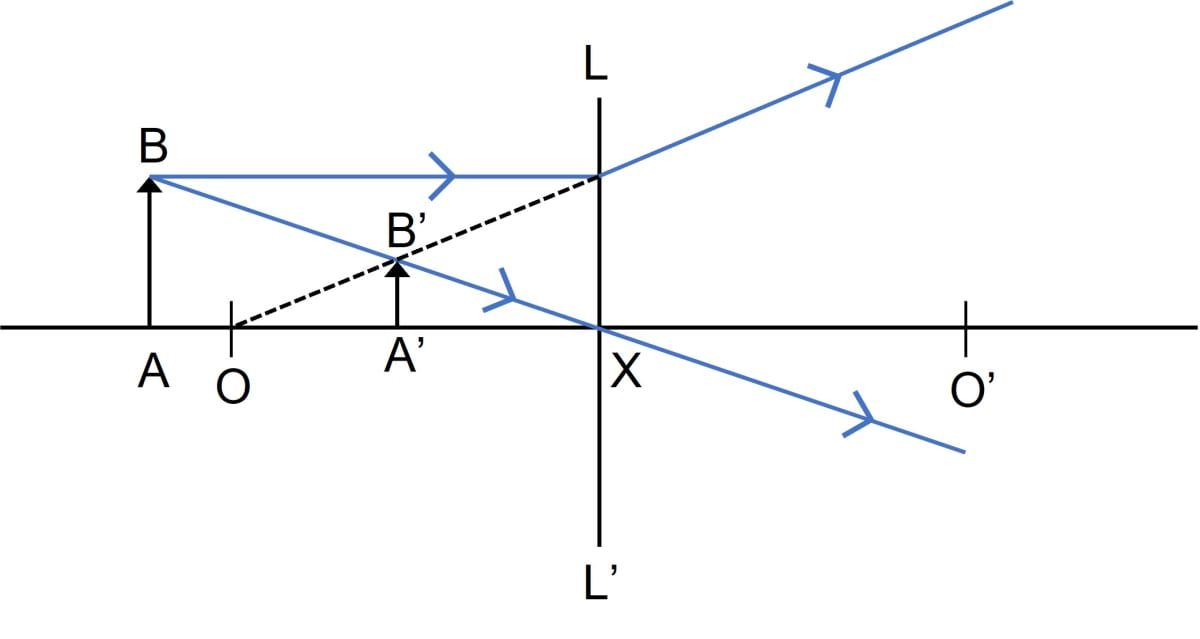(iv) The three characteristics of the image are virtual, erect and diminished.

#### Question 7

The following diagram in figure shows an object AB and a converging lens L with foci F1 and F2.

(a) Draw two rays from the object AB and complete the diagram to locate the position of the image CD. Also mark on the diagram the position of eye from where the image can be viewed.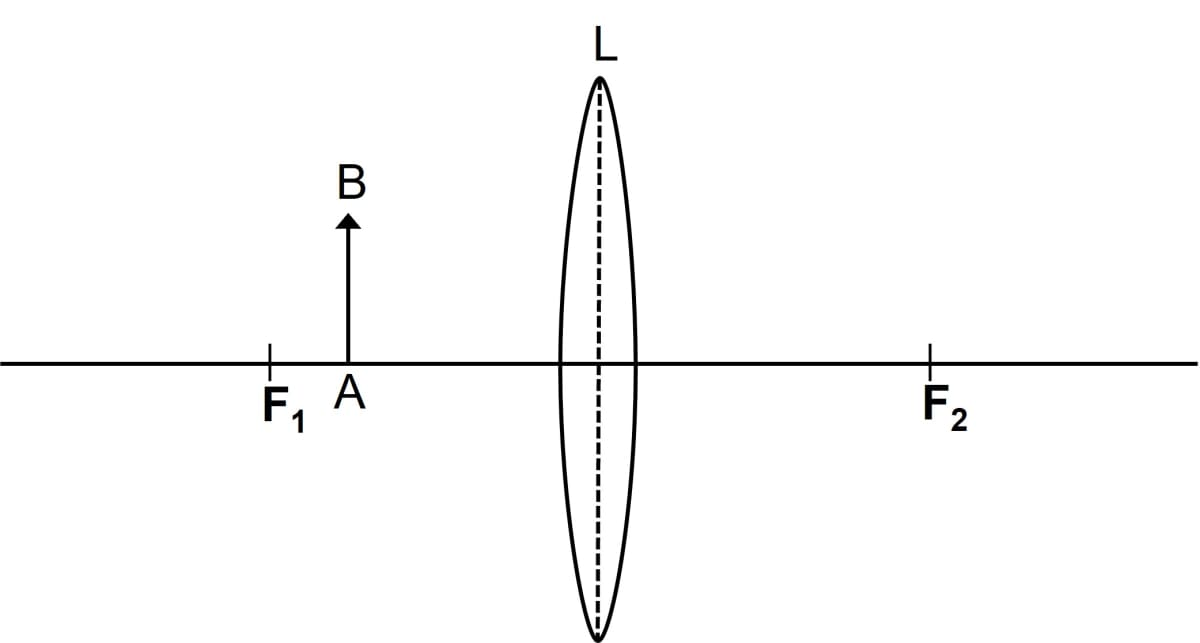(b) State three characteristics of the image in relation to the object.

(a) Below is the completed diagram showing the position of the image CD and the position of eye from where the image can be viewed:(b) The three characteristics of the image are magnified, virtual and upright.

#### Question 8

The diagram given below in figure shows the position of an object OA in relation to a converging lens L whose foci are at F1 and F2.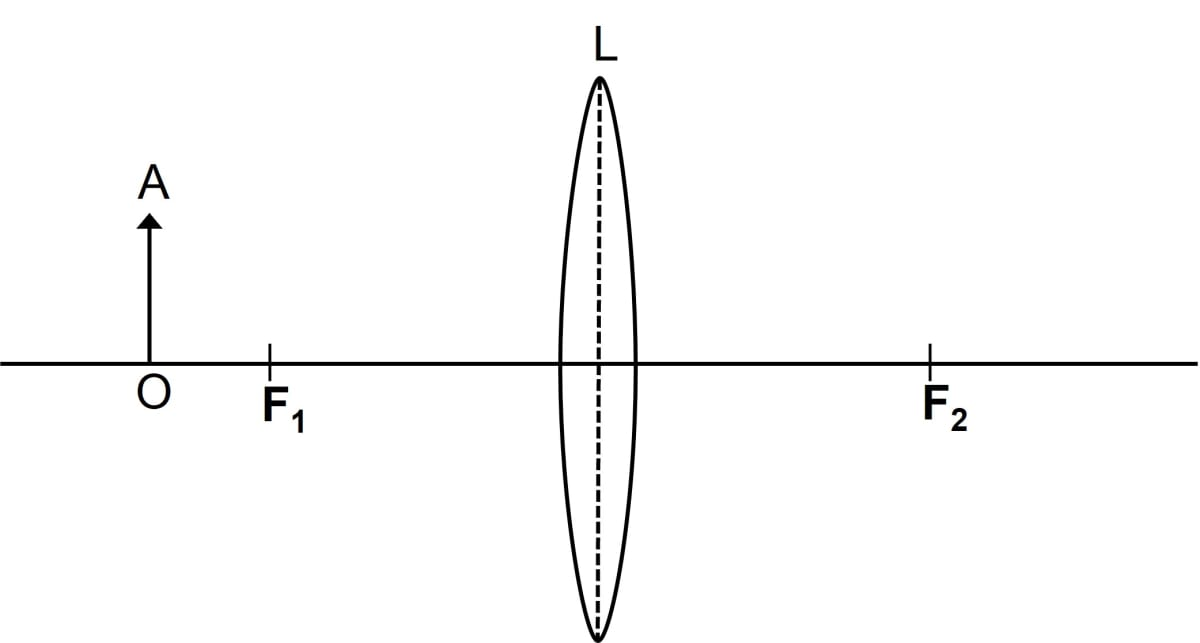(i) Draw two rays to locate the position of the image.

(ii) State the position of the image with reference to the lens.

(iii) Describe the three characteristics of the image.

(iv) Describe how the distance of the image from the lens and its size change as the object is moved towards F1.

(i) Below ray diagram shows the position of the image: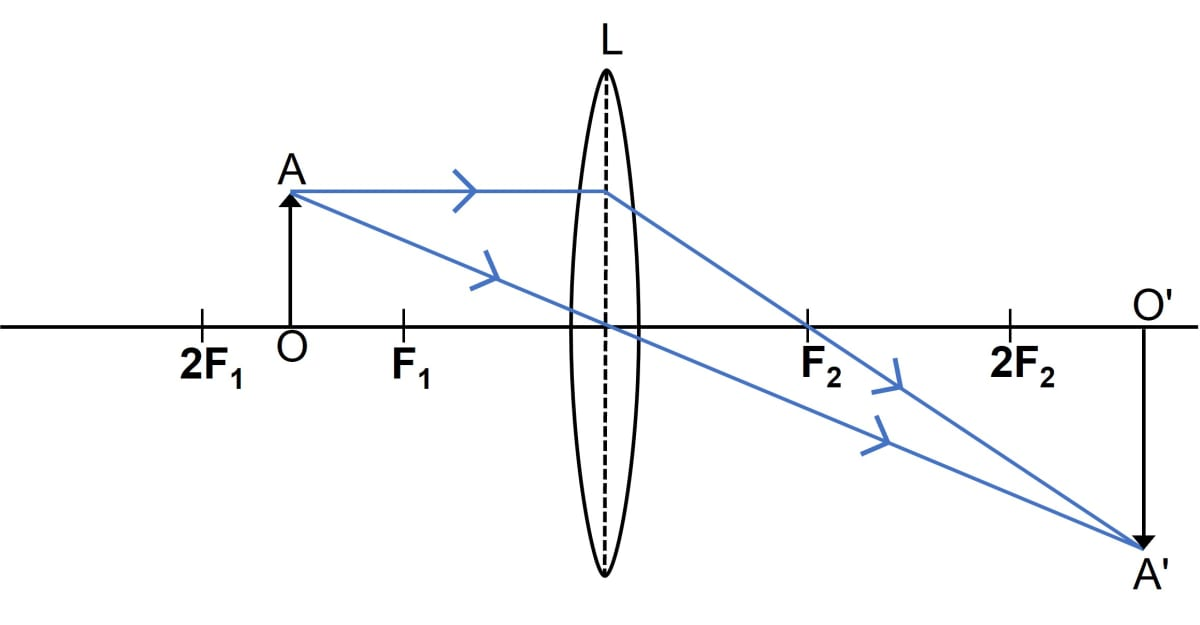(ii) Position of images will be more than twice the focal length of the lens.

(iii) The three characteristics of this image are magnified, real and inverted.

(iv) When the object move towards F1, the image will shift away from F2 and it will be magnified.

The image will form at infinity and it is highly magnified at F1.

Between F1 and optical centre, the image will form on the same side of the object and will be magnified.

#### Question 9

A converging lens forms the image of an object placed in front of it, beyond 2F2 of the lens.

(a) Where is the object placed?

(b) Draw a ray diagram to show the formation of an image.

(c) State three characteristics of the image.

(a) The object is placed between F1 and 2F1.

(b) Below is the ray diagram showing an object placed between F1 and 2F1 of the lens and its image is formed beyond 2F2 of the lens: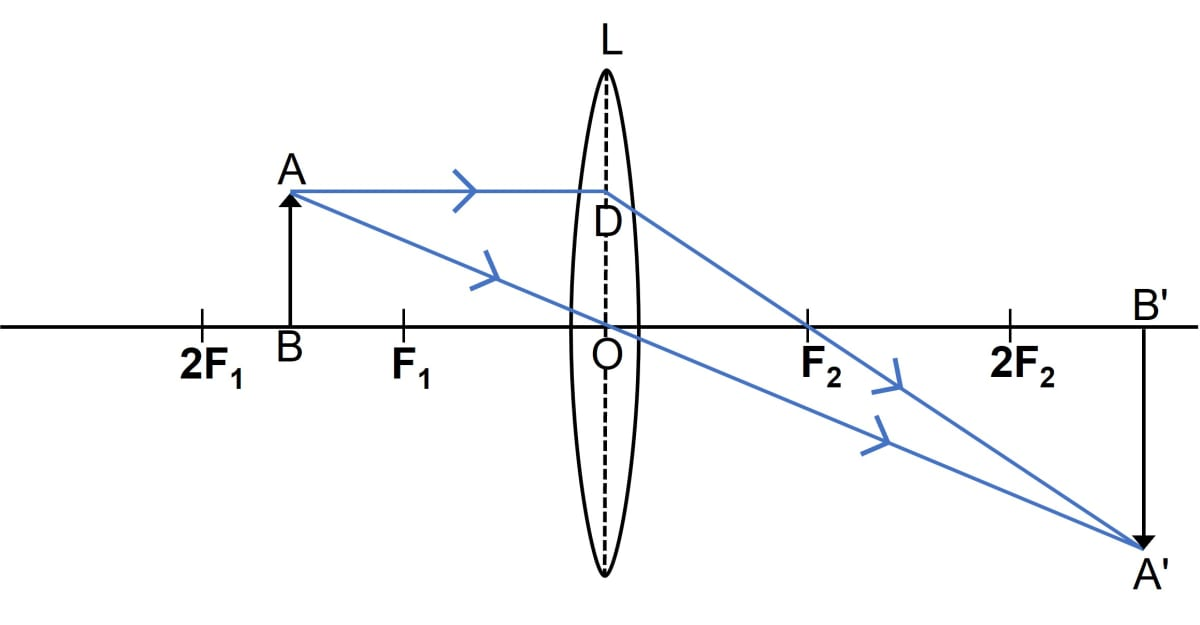(c) The three characteristics of the image is real, inverted and magnified.

#### Question 10

A convex lens forms an image of an object equal to the size of the object.

(a) Where is the object placed in front of the lens?

(b) Draw a diagram to illustrate it.

(c) State two more characteristics of the image.

(a) The object is placed at a distance equal to twice the focal length of the lens (i.e., Object is placed at 2F1).

(b) Below diagram illustrates the formation of an image equal in size of the object by a convex lens: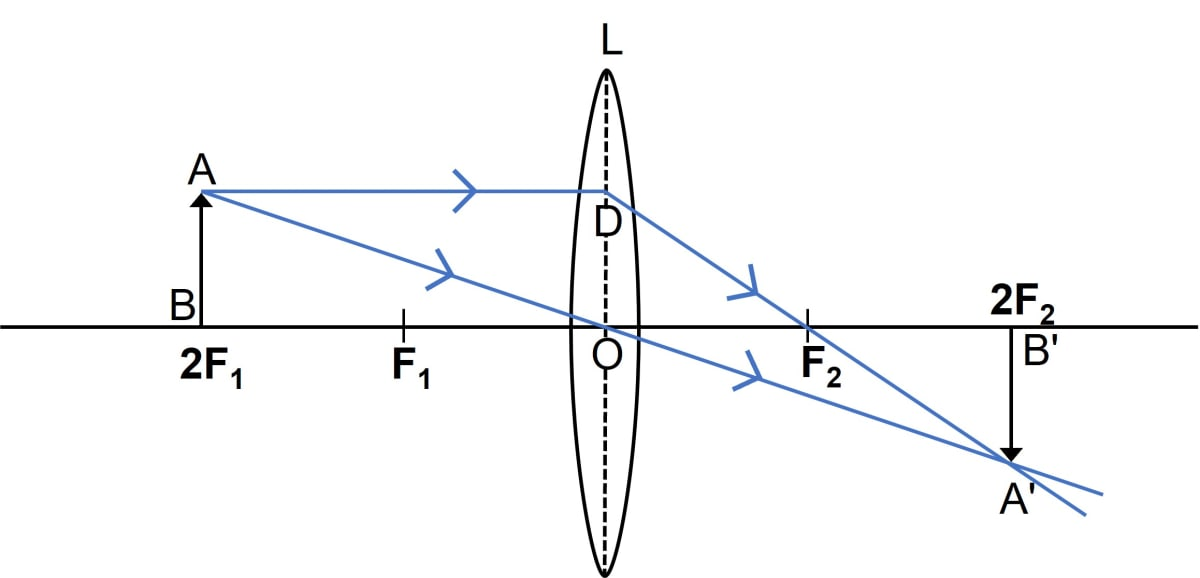(c) The image formed is real and inverted

#### Question 11

A lens forms an erect, magnified and virtual image of an object.

(a) Name the kind of lens.

(b) Where is the object placed in relation to the lens?

(c) Draw a ray diagram to show the formation of image.

(d) Name the device which uses this principle.

(a) When a lens forms an erect, magnified and virtual image of an object then we can say that it is a convex lens.

(b) The object is placed between the lens and focus (F1)

(c) Below ray diagram shows the formation of the image: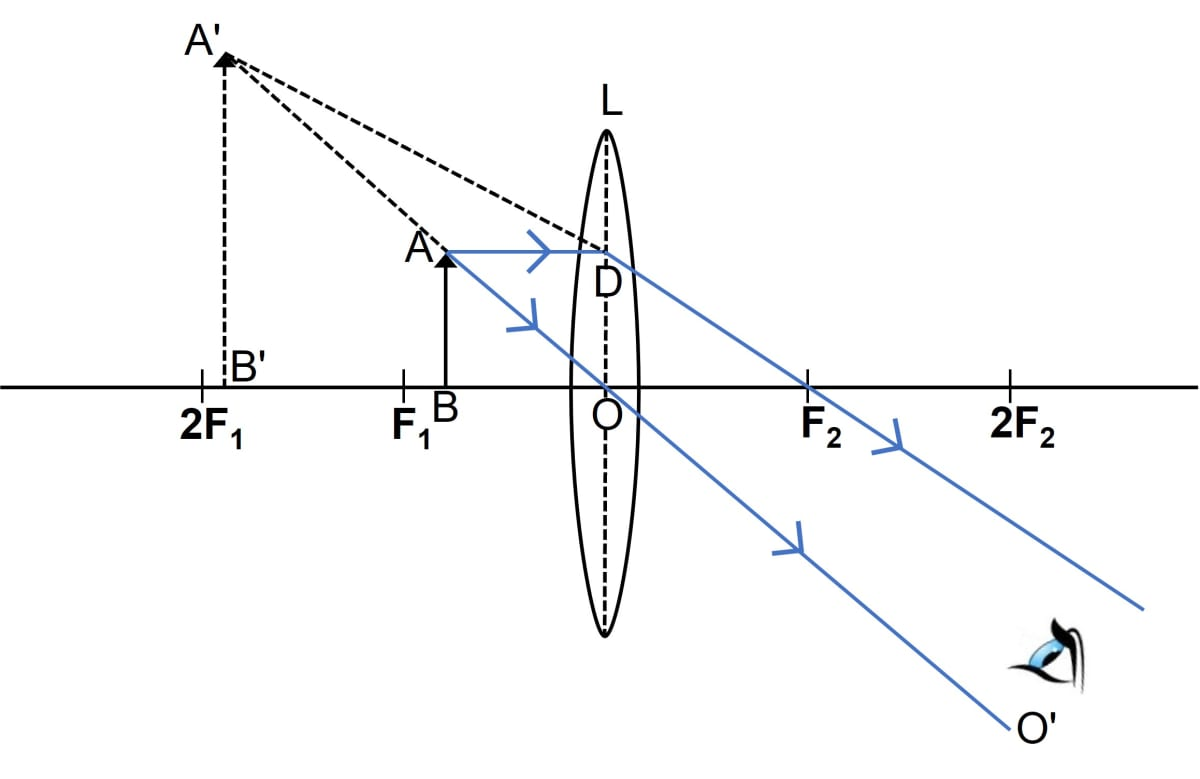(d) This principle is used in magnifying glass.

#### Question 12

A lens forms an image between the object and the lens.

(a) Name the lens.

(b) Draw a ray diagram to show the formation of such an image.

(c) State three characteristics of the image.

A lens forms an image between the object and the lens. Name the lens. Draw a ray diagram to show the formation of such an image. State three characteristics of the image.

(a) When a lens forms an image between the object and the lens then we can say that it is a concave lens.

(b) Below ray diagram shows the formation of the image: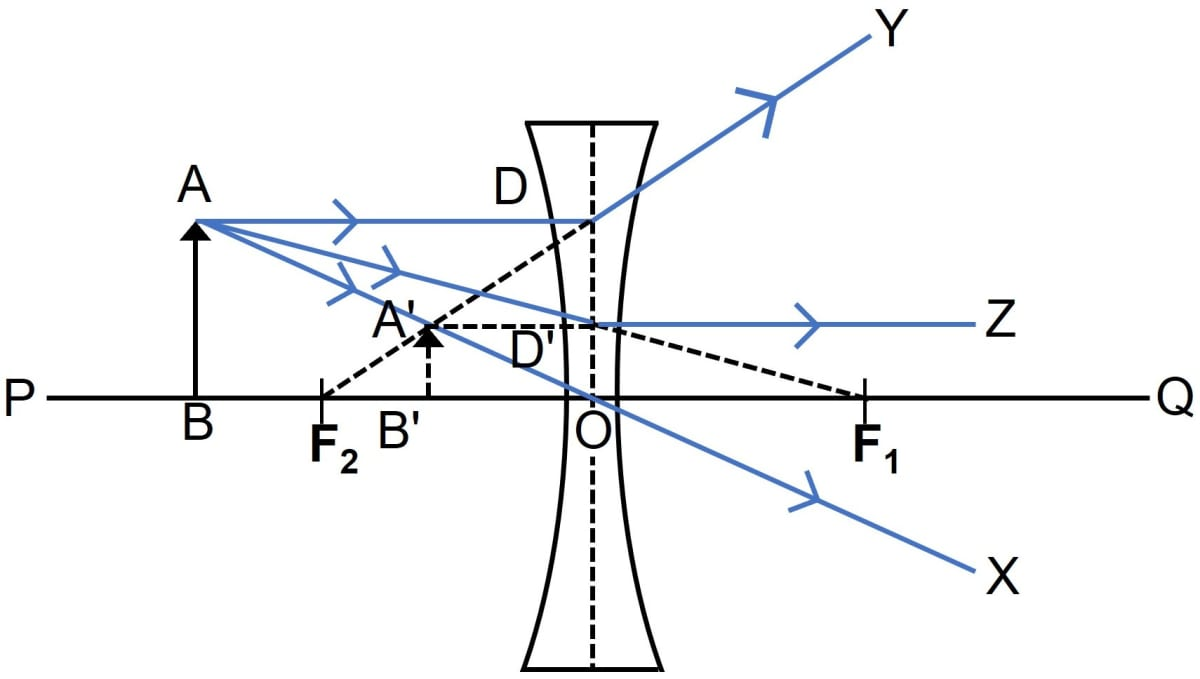(c) The three characteristics of the image are virtual, erect and diminished.

#### Question 13

Classify as real or virtual, the image of a candle flame formed on a screen by a convex lens. Draw a ray diagram to illustrate how an image is formed.

When the candle is placed beyond 2F1, the image is formed between F2 and 2F2.

The image is real, diminished and inverted.

In the ray diagram shown below, the candle is represented as AB and its real, inverted and diminished image is represented as A'B' formed between F2 and 2F2.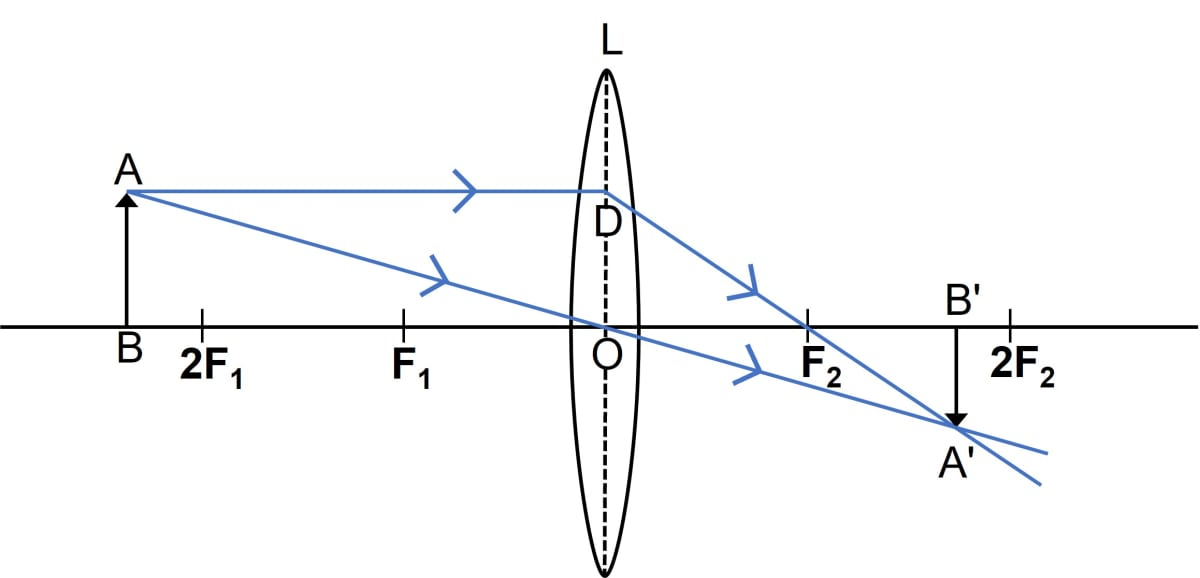#### Question 14

Show by a ray diagram that a diverging lens cannot form a real image of an object placed anywhere on its principal axis.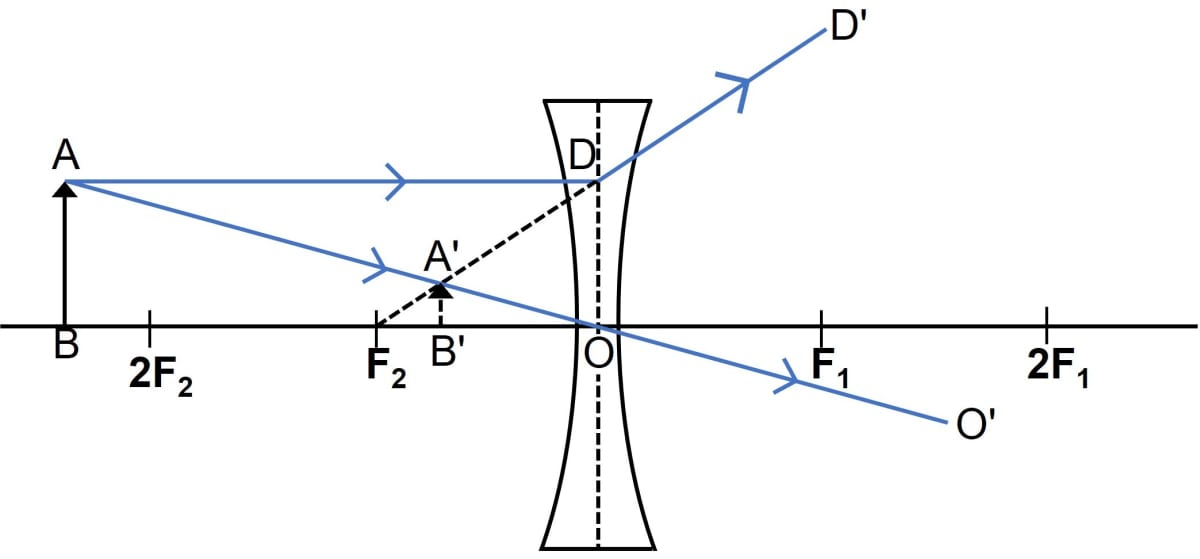When the object is at any finite distance from the concave lens, the image formed is virtual, erect and diminished. Hence, a diverging lens (concave lens) cannot form a real image.

#### Question 15

Draw a ray diagram to show how a converging lens can form a real and enlarged image of an object.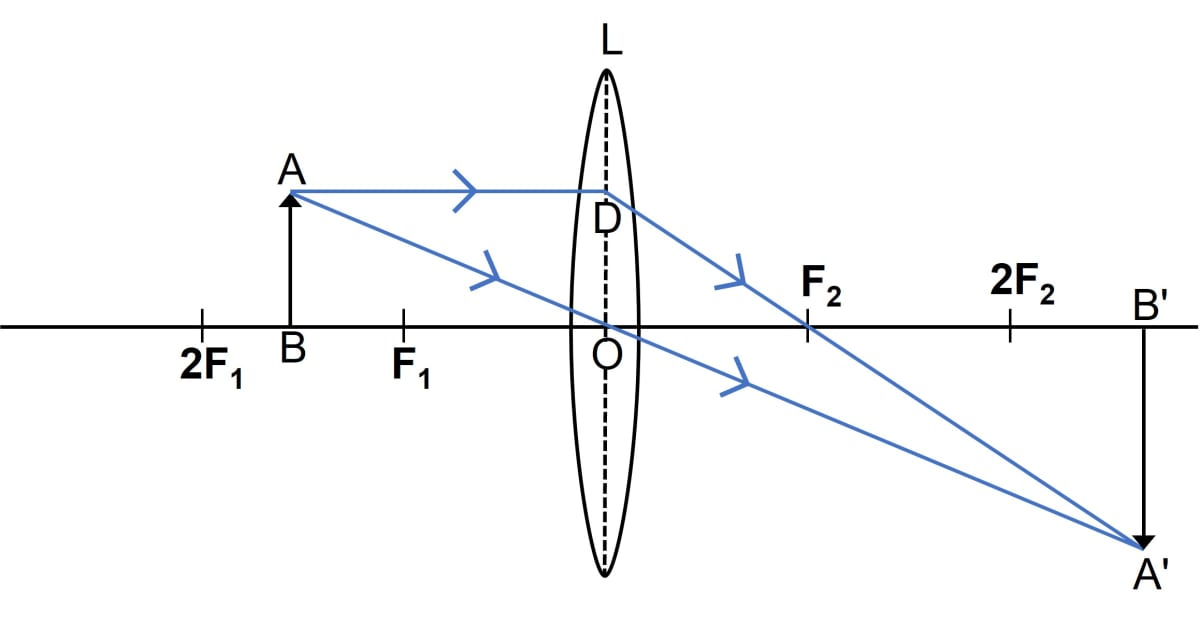When the object is placed between 2F1 and F1 then the image is formed beyond 2F2.

The image is real, enlarged and inverted.

#### Question 16

A lens forms an upright and diminished image of an object placed at its focal point. Name the lens and draw a ray diagram to show the formation of an image.

It is a concave lens.

Below ray diagram shows the formation of an image by a concave lens: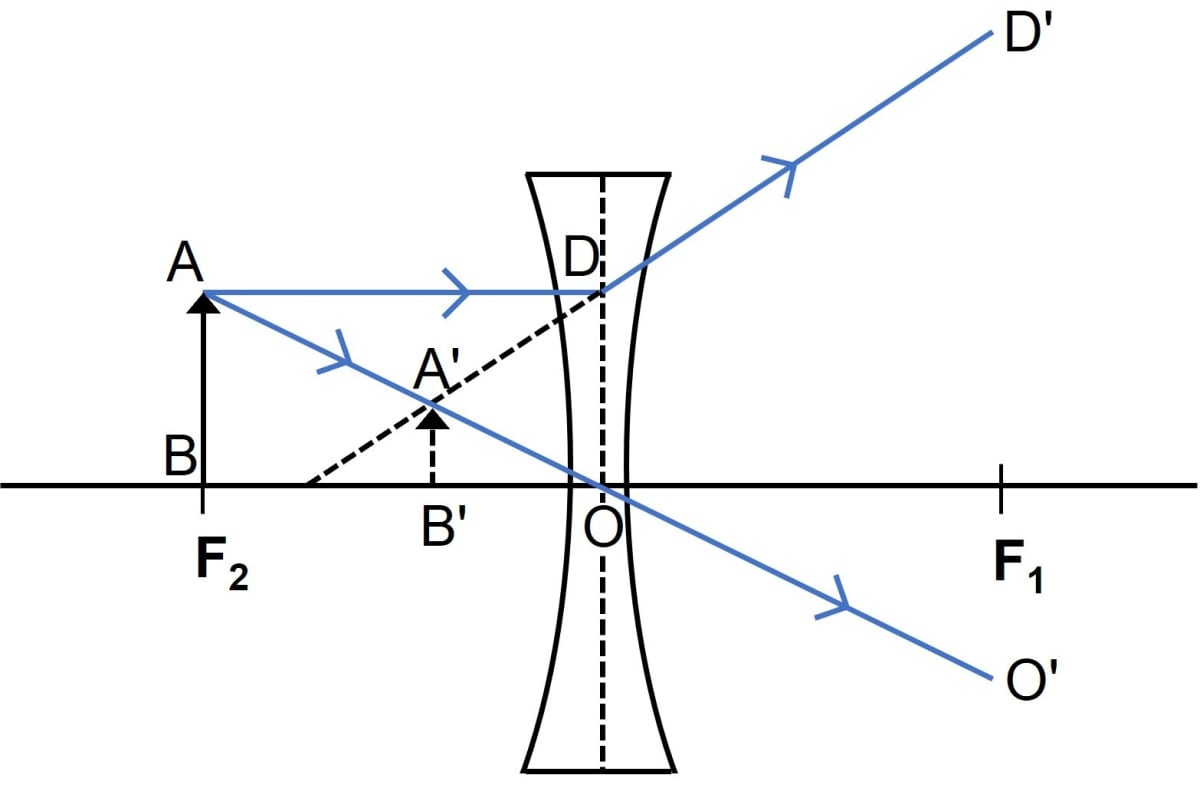#### Question 17

Draw a ray diagram to show how a converging lens is used as a magnifying glass to observe a small object. Mark on your diagram the foci of the lens and the position of the eye.When the object is placed between the convex lens and focal point F1 then the image is formed on the same side of the lens. The image so formed is enlarged.

As the image formed is enlarged so we can say that the converging lens can be used as a magnifier.

#### Question 18

Draw a ray diagram to show how a converging lens can form an image of the Sun. Hence give a reason for the term 'burning glass' for a converging lens used in this manner.

Below ray diagram shows how a converging lens can form an image of the Sun: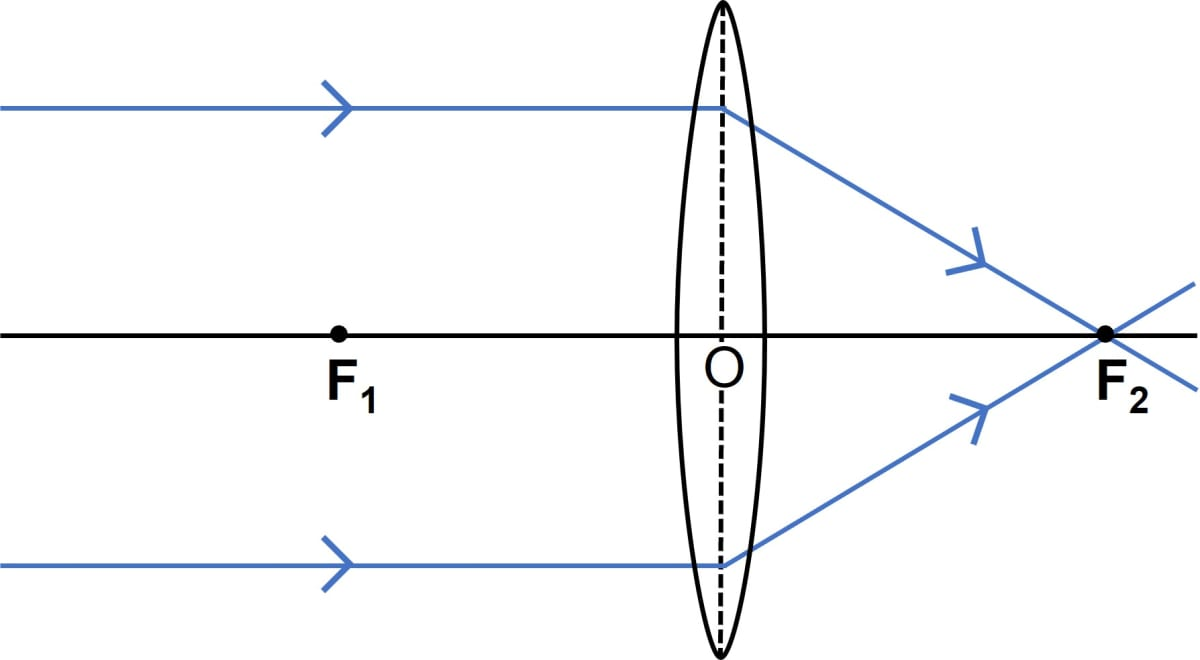The Sun is at infinity so convex lens forms its image at the second focal point which is real and very much diminished in size.

The rays of light from the sun converge to a single point called the focus of the lens. This concentrated image of the Sun burns the paper kept below the lens. Hence, the term burning glass is used for the lens.

#### Question 19

A lens forms an inverted image of an object.

(a) Name the kind of lens.

(b) State the nature of the image whether real or virtual?

(a) It is a convex lens as a convex lens forms an inverted image when the object is placed anywhere from infinity to F1.

(b) The image formed by a convex lens is real.

#### Question 20

A lens forms an upright and magnified image of an object.

(a) Name the lens.

(b) Draw a labelled ray diagram to show the image formation.

(a) The lens is a convex lens. A convex lens forms an upright and magnified image, when the position of the object is between the lens and F1.

(b) Below labelled ray diagram shows the image formation:#### Question 21

(a) Name the lens which always forms an erect and virtual image.

(b) State whether the image in part (a) is magnified or diminished?

(a) A concave lens always forms an erect and virtual image.

(b) The image formed by a concave lens is always diminished.

#### Question 22

Can a concave lens form an image of size two times that of the object? Give reason?

No, a concave lens cannot form an image of size of two times that of the object as a concave lens diverges the rays incident on it, and the image is always diminished.

#### Question 23

Give two characteristics of the image formed by a concave lens.

A concave lens diverges the rays incident on it, and so the image formed by a concave lens is always virtual and diminished.

#### Question 24

Give two characteristics of the virtual image formed by a convex lens.

The virtual image formed by a convex lens will be erect and magnified.

#### Question 25

In each of the following cases, where must an object be placed in front of a convex lens so that the image formed is,

(a) at infinity,

(b) of same size as the object,

(c) inverted and enlarged,

(d) upright and enlarged?

(a) In order to form the image at infinity, the object should be placed at focus, in front of a convex lens.

(b) In order to form the image of same size as the object, the object should be placed at 2F, in front of a convex lens.

(c) In order to form an inverted and enlarged image, the object should be placed between F and 2F, in front of a convex lens.

(d) In order to form an upright and enlarged image, the object should be placed between optical centre and focus, in front of a convex lens.

#### Question 26

Complete the following table —

Type of lensPosition of objectNature of imageSize of image
ConvexBetween the optical centre and focus
ConvexAt focus
ConcaveAt infinity
ConcaveAt any distance

Type of lensPosition of objectNature of imageSize of image
ConvexBetween the optical centre and focusVirtual and uprightMagnified
ConvexAt focusReal and invertedHighly magnified
ConcaveAt infinityVirtual and uprightHighly diminished
ConcaveAt any distanceVirtual and uprightDiminished

#### Question 27

State the changes in the position, size and nature of the image when the object is brought from infinity up to the convex lens. Illustrate your answer by drawing the ray diagrams.

(i) When the object is situated at infinity, the position of the image is at F2. The image is real and inverted. It is highly diminished in size.(ii) When the object is situated beyond 2F1, the position of the image is between F2 and 2F2. The image is real and inverted. It is diminished in size.(iii) When the object is situated at 2F1, the position of the image is at 2F2. The image is real and inverted. It is of the same size as the object.(iv) When the object is situated between 2F1 and F1, the position of the image is beyond 2F2. The image is real and inverted. It is magnified in size.(v) When the object is situated at F1, the position of the image is at infinity. The image is real and inverted. It is highly magnified in size.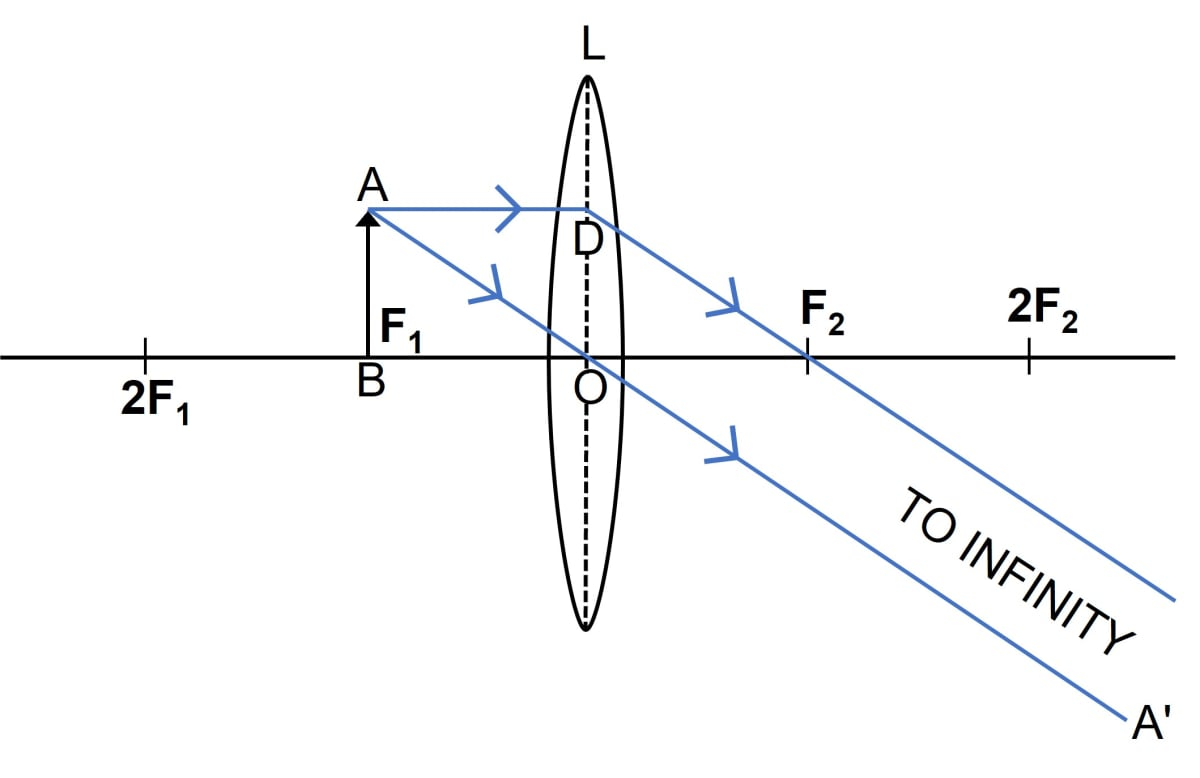(vi) When the object is situated between lens and F1, the position of the image is on the same side . The image is virtual and upright. It is magnified in size.#### Question 28

State the changes in the position, size and nature of the image when the object is brought from infinity up to the concave lens. Illustrate your answer by drawing ray diagrams.

(i) When the object is at infinity, the image is formed at the second focus F2, on the side of the object. It is virtual and erect. It is highly diminished in size.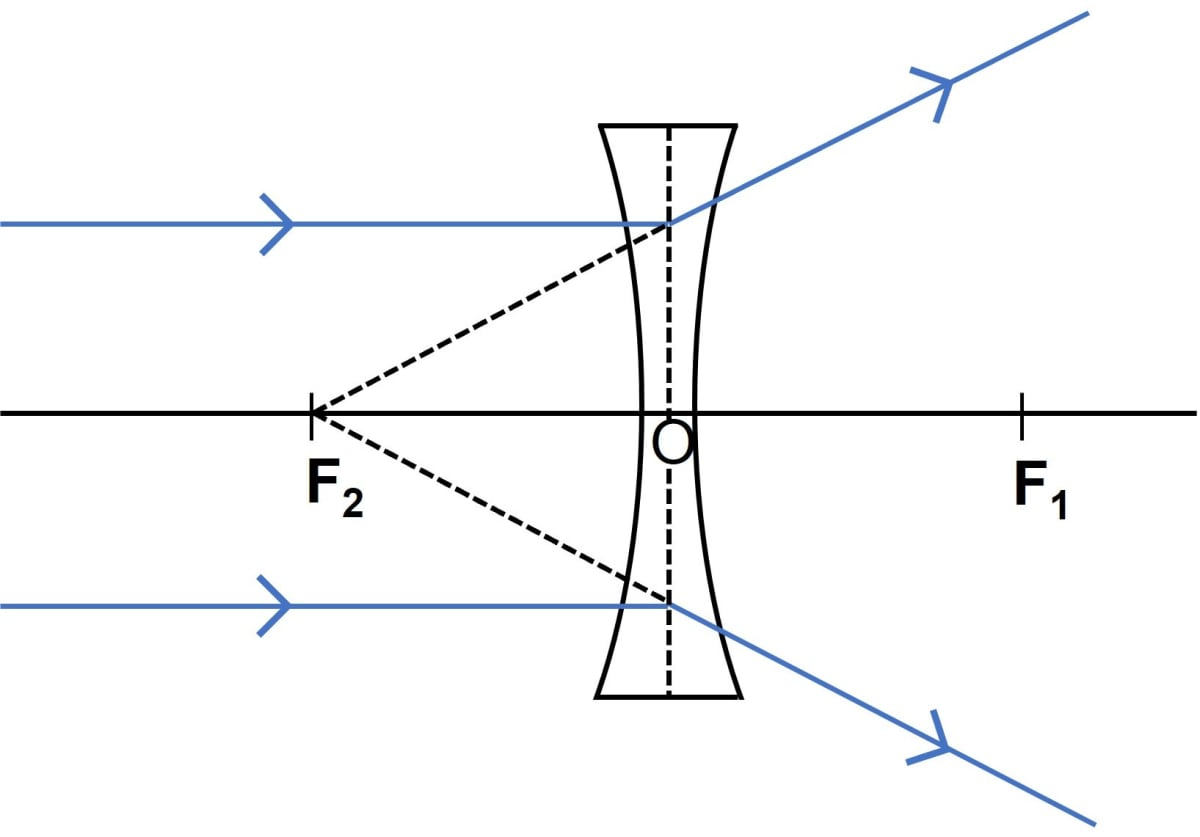(ii) When the object is at finite distance from the concave lens, the image is formed between the focus and optical centre, on the same side of lens as the object. It is virtual and erect. It is diminished in size.#### Question 29

Complete the following sentences —

(a) An object is placed at a distance of more than 40 cm from a convex lens of focal length 20 cm. The image formed is real, inverted and …………….

(b) An object is placed at a distance 2f from a convex lens of focal length f. The size of the image formed is …………… that of the object

(c) An object is placed at a distance 5 cm from a convex lens of focal length 10 cm. The image formed is virtual, upright and …………..

(a) An object is placed at a distance of more than 40 cm from a convex lens of focal length 20 cm. The image formed is real, inverted and diminished.

(b) An object is placed at a distance 2f from a convex lens of focal length f. The image formed is equal to that of the object.

(c) An object is placed at a distance 5 cm from a convex lens of focal length 10 cm. The image formed is virtual, upright and magnified.

## State 'true' or 'false'

#### Question 30(a)

A convex lens has a divergent action and a concave lens has a convergent action.
False

#### Question 30(b)

A concave lens, if kept at a proper distance from an object, can form its real image.
False

#### Question 30(c)

A ray of light incident parallel to the principal axis of a lens, passes undeviated after refraction.
False

#### Question 30(d)

A ray of light incident at the optical centre of a lens, passes undeviated after refraction.
True

#### Question 30(e)

A concave lens forms a magnified or diminished image depending on the distance of an object from it.
False

## Multiple Choice Type

#### Question 1

For an object placed at a distance 20 cm in front of a convex lens, the image is at a distance 20 cm behind the lens. The focal length of the convex lens is:

1. 20 cm
2. 10 cm ✓
3. 15 cm
4. 40 cm

Given, object distance = image distance.

u = 20 cm

Hence, object must be at 2f.

Therefore,

$u = 2f \\[0.5em]$

Substituting the value of u in the equation above, we get,

$20 = 2f \\[0.5em] \Rightarrow f = \dfrac{20}{2} \\[0.5em] \Rightarrow f = 10 cm \\[0.5em]$

#### Question 2

For the object placed between the optical centre and focus of a convex lens, the image is:

1. real and enlarged
2. real and diminished
3. virtual and enlarged ✓
4. virtual and diminished.

Whenever, the object is placed between the optical centre and focus of a convex lens, the image is virtual, erect and magnified.

We can understand this better with the help of the diagram given below.#### Question 3

A concave lens forms the image of an object which is:

1. virtual, inverted and diminished
2. virtual, upright and diminished ✓
3. virtual, inverted and enlarged
4. virtual, upright and enlarged

Irrespective of the position of the object, a concave lens always forms an image which is virtual, upright and diminished and it is situated on the side of the object between the focus and the lens.

## Exercise 5(C)

#### Question 1

State the sign convention to measure the distances for a lens.

We follow the cartesian sign convention to measure the distance in a lens according to which:

1. The optical centre of the lens is chosen as the origin of the coordinate system.
2. The object is considered to be placed on the principle axis to the left of the lens.
3. All the distances are measured along the principle axis from the optical centre of the lens. The distance of the object from the lens is denoted by u, the distance of the image by v and the distance of the second focus by f.
4. The distances measured in the direction of the incident ray are taken positive, while the distances opposite to the direction of incident ray are taken negative.
5. The length above the principle axis is taken positive, while the length below the principle axis is taken negative.
6. By sign convention, the focal length of the convex lens is positive and that of the concave lens is negative.
7. The distance of object (u) infront of lens is always negative. The distance of image (v) is positive if it is real and formed behind the lens, while it is negative if the image is virtual and formed in front of the lens.

#### Question 2

The focal length of a lens is (i) positive, (ii) negative. In each case, state the kind of lens.

(i) When the focal length of a lens is positive, then the lens is a convex lens.

(ii) When the focal length of a lens is negative, then the lens is a concave lens.

#### Question 3

Write the lens formula explaining the meaning of the symbols used.

Lens formula,

$\dfrac{1}{v} – \dfrac{1}{u} = \dfrac{1}{f}$

where, 'u' is the distance of the object from the optical centre of the lens, 'v' is the distance of the image from the optical centre while 'f' is the focal length, the distance between the optical centre and the focus of the lens.

#### Question 4

What do you understand by the term magnification? Write an expression for it for a lens, explaining the meaning of the symbols used.

The ratio of length of image I perpendicular to the principal axis, to the length of the object O, is called linear magnification.

The expression is:

$m = \dfrac{\text{length of image (I)}}{\text{length of object (O)}} = \dfrac{v}{u} \\[0.5em]$

where,

v = image distance

u = object distance.

#### Question 5

What information about the nature of the image (i) real or virtual, (ii) erect or inverted, do you get from the sign of magnification + or - ?

(i) The positive sign of magnification indicates a virtual image while a negative sign indicates a real image.

(ii) The positive sign of magnification indicate an erect image while a negative sign indicates an inverted image.

#### Question 6

Define the term power of a lens. In what unit is it expressed ?

The deviation of the incident light rays produced by a lens on refraction through it is a measure of its power.

The S.I. unit of power is dioptre (D).

#### Question 7

How is the power of a lens related to its focal length ?

The relation between power of a lens and its focal length is as follows —

$P = \dfrac{1}{f}$

Where,

P = power of lens and

f = focal length of lens.

Hence, power of a lens is inversely proportional to its focal length.

#### Question 8

How does the power of a lens change if its focal length is doubled?

As we know that,

$P = \dfrac{1}{f}$

Where,

P = power of lens and

f = focal length of lens.

Hence, power of a lens is inversely proportional to its focal length.

$P = \dfrac{1}{2f}$

So, if the focal length is doubled, the power gets halved.

#### Question 9

How is the sign (+ or -) of power of a lens related to its divergent or convergent action?

Depending on the direction in which a lens deviates the light rays, its power is either positive or negative.

If a lens deviates a ray towards its centre (converges), the power is positive and if it deviates the ray away from its centre (diverges), the power is negative.

Hence, the power of a convex lens is positive and of a concave lens is negative.

#### Question 10

The power of a lens is negative. State whether it is convex or concave?

It is a concave lens

#### Question 11

Which lens has more power : a thick lens or a thin lens ?

When comparison is done between a thick lens and a thin lens then, a thick lens has more power than a thin lens.

A thick lens (i.e. a lens having surfaces of more curvature) is of short focal length and it deviates the rays more, while a thin lens (i.e. a lens having surfaces of less curvature) is of large focal length and deviates the rays less as power of a lens is reciprocal of its focal length.

## Multiple Choice Type

#### Question 1

If the magnification produced by a lens is –0.5, the correct statement is —

1. the lens is concave
2. the image is virtual
3. the image is magnified
4. the image is real and diminished formed by a convex lens ✓

The magnification produced by a lens is –0.5, which shows a negative sign. The negative sign of magnification indicates that the image is real while 0.5 indicates that the image is diminished.

#### Question 2

The correct lens formula is

1. $\dfrac{1}{u} + \dfrac{1}{v} = \dfrac{1}{f}$
2. $\dfrac{1}{u} - \dfrac{1}{v} = \dfrac{1}{f}$
3. $\bold{\dfrac{1}{v}} \bold{-} \bold{\dfrac{1}{u}} = \bold{\dfrac{1}{f}}$
4. $f = \dfrac{(u + v)}{uv}$

The correct lens formula is

$\dfrac{1}{v} - \dfrac{1}{u} = \dfrac{1}{f}$

#### Question 3

On reducing the focal length of a lens, its power:

1. Decreases
2. Increases ✓
3. Does not change
4. First increases then decreases.

As we know that,

$P = \dfrac{1}{f}$

Where,

P = power of lens and

f = focal length of lens.

Hence, power of a lens is inversely proportional to its focal length.

So, on reducing the focal length of a lens, its power increases.

#### Question 4

The lens of power +1.0 D is :

1. convex of focal length 1.0 cm
2. convex of focal length 1.0 m ✓
3. concave of focal length 1.0 cm
4. concave of focal length 1.0 m

As we know that,

$P = \dfrac{1}{f}$

Where,

P = power of lens and

f = focal length of lens.

Given,

power = + 1.0 D

Substituting the values in the formula we get,

$+1.0 D = \dfrac{1}{fm} \\[0.5em] \Rightarrow f = 1.0m$

Hence, focal length = 1.0 m

## Numericals

#### Question 1

(a) At what position a candle of length 3 cm be placed in front of a convex lens so that its image of length 6 cm be obtained on a screen placed at a distance 30 cm behind the lens?

(b) What is the focal length of the lens in part (a)?

(a) As we know,

The formula for magnification of a lens is —

$m = \dfrac{\text{length of image (I)}}{\text{length of object (O)}} = \dfrac{v}{u} \\[0.5em]$

where,

v = image distance

u = object distance.

Given,

Height of a candle, O = 3 cm

Height of the image of candle, I = 6 cm

Image distance, v = 30 cm

Substituting the values in the formula we get,

$\dfrac{6}{3} = \dfrac{30}{u} \\[0.5em] u = \dfrac{30 \times 3}{6} \\[0.5em] u = 5 \times 3 \\[0.5em] \Rightarrow u = 15$

Therefore, object distance is equal to 15 cm.

(b) As we know, lens formula is —

$\dfrac{1}{v} – \dfrac{1}{u} = \dfrac{1}{f} \\[0.5em]$

Substituting the values in the formula we get,

$\dfrac{1}{30} – \dfrac{1}{-15} = \dfrac{1}{f} \\[0.5em] \dfrac{1}{30} + \dfrac{1}{15} = \dfrac{1}{f} \\[0.5em] \dfrac{2 + 1}{30} = \dfrac{1}{f} \\[0.5em] \dfrac{3}{30} = \dfrac{1}{f} \\[0.5em] f = \dfrac{30}{3} \\[0.5em] \Rightarrow f = 10$

Hence, the focal length of the lens, f = 10 cm.

#### Question 2

A concave lens forms the image of an object kept at a distance 20 cm in front of it, at a distance 10 cm on the side of the object.

(a) What is the nature of the image?

(b) Find the focal length of the lens.

(a) As the lens used is a concave lens, so the image formed will be erect and diminished. It will be virtual in nature as the image is formed on the same side as the object.

(b) As we know, the lens formula is —

$\dfrac{1}{v} – \dfrac{1}{u} = \dfrac{1}{f} \\[0.5em]$

Given

u = -20 cm

v = – 10 cm

Substituting the values in the formula we get,

$\dfrac{1}{-10} – \dfrac{1}{-20} = \dfrac{1}{f} \\[0.5em] -\dfrac{1}{10} + \dfrac{1}{20} = \dfrac{1}{f} \\[0.5em] \dfrac{-2 + 1}{20} = \dfrac{1}{f} \\[0.5em] -\dfrac{1}{20} = \dfrac{1}{f} \\[0.5em] \Rightarrow f = -20 cm$

Therefore, focal length of the lens = 20 cm (negative)

#### Question 3

The focal length of a convex lens is 25 cm. At what distance from the optical centre of the lens an object be placed to obtain a virtual image of twice the size ?

As we know,

The formula for magnification of a lens is —

$m = \dfrac{v}{u} \\[0.5em]$

Given,

f = + 25 cm

As image is virtual and magnified, so m = + 2

Substituting the values in the formula we get,

$+2 = \dfrac{v}{u} \\[0.5em] \Rightarrow v = 2u \\[0.5em]$

Therefore, we get, v = 2u

Now as we know, the lens formula is —

$\dfrac{1}{v} – \dfrac{1}{u} = \dfrac{1}{f} \\[0.5em]$

Substituting the values in the formula, we get,

$\dfrac{1}{2u} – \dfrac{1}{u} = \dfrac{1}{25} \\[0.5em] \dfrac{1-2}{2u} = \dfrac{1}{25} \\[0.5em] -\dfrac{1}{2u} = \dfrac{1}{25} \\[0.5em] 2u = - 25 \\[0.5em] \Rightarrow u = - 12.5 cm \\[0.5em]$

Therefore, object should be placed at a distance of 12.5 cm infront of the lens.

#### Question 4

Where should an object be placed in front of a convex lens of focal length 0.12 m to obtain a real image of size three times the size of the object, on the screen?

(i) As we know,

The formula for magnification of a lens is —

$m = \dfrac{v}{u} \\[0.5em]$

Given,

f = +0.12 m

Image is real and magnified, m = -3

Substituting the values in the formula we get,

$-3 = \dfrac{v}{u} \\[0.5em] \Rightarrow v = -3u \\[0.5em]$

Therefore, we get, v = -3u

Now as we know, the lens formula is —

$\dfrac{1}{v} – \dfrac{1}{u} = \dfrac{1}{f} \\[0.5em]$

Substituting the values in the formula, we get,

$\dfrac{1}{-3u} – \dfrac{1}{u} = \dfrac{1}{0.12} \\[0.5em] \dfrac{-1-3u}{3u} = \dfrac{1}{0.12} \\[0.5em] -\dfrac{4}{3u} = \dfrac{1}{0.12}\\[0.5em] u = - \dfrac{4 \times 0.12}{3} \\[0.5em] \Rightarrow u = - 0.16 m \\[0.5em]$

Therefore, object should be placed at a distance of 0.16 m infront of the lens.

#### Question 5

An illuminated object lies at a distance 1.0 m from a screen. A convex lens is used to form the image of the object on a screen placed at a distance of 75 cm from the lens.

Find —

(i) the focal length of lens, and

(ii) the magnification.

As we know, the lens formula is —

$\dfrac{1}{v} – \dfrac{1}{u} = \dfrac{1}{f} \\[0.5em]$

Given,

Object lies at a distance 1.0 m from a screen.

v = 75 cm

So, u = - 25 cm

Substituting the values in the formula, we get,

$\dfrac{1}{75} – \dfrac{1}{-25} = \dfrac{1}{f} \\[0.5em] \dfrac{1}{75} + \dfrac{1}{25} = \dfrac{1}{f} \\[0.5em] \dfrac{1+3}{75} = \dfrac{1}{f} \\[0.5em] \dfrac{4}{75} = \dfrac{1}{f}\\[0.5em] \Rightarrow f = \dfrac{75}{4} \\[0.5em] \Rightarrow f = \text{18.75 cm} \\[0.5em]$

Therefore, focal length of the lens is 18.75cm.

ii) As we know,

the formula for magnification of a lens is —

$m = \dfrac{v}{u} \\[0.5em]$

Given,

v = 75 cm

u = - 25 cm

Substituting the values in the formula, we get,

$m = \dfrac{75}{-25} \\[0.5em] m = -3 \\[0.5em]$

Therefore, the magnification is -3.

#### Question 6

A lens forms the image of an object placed at a distance 15 cm from it, at a distance 60 cm in front of it.

Find —

(i) the focal length,

(ii) the magnification, and

(iii) the nature of image.

As we know, the lens formula is —

$\dfrac{1}{v} – \dfrac{1}{u} = \dfrac{1}{f} \\[0.5em]$

Given,

v = -60 cm

So, u = -15 cm

Substituting the values in the formula, we get,

$\dfrac{1}{-60} – \dfrac{1}{-15} = \dfrac{1}{f} \\[0.5em] - \dfrac{1}{60} + \dfrac{1}{15} = \dfrac{1}{f} \\[0.5em] \dfrac{1}{15} - \dfrac{1}{60} = \dfrac{1}{f} \\[0.5em] \dfrac{4-1}{60} = \dfrac{1}{f} \\[0.5em] \dfrac{3}{60} = \dfrac{1}{f}\\[0.5em] \Rightarrow f = \dfrac{60}{3} \\[0.5em] \Rightarrow f = 20cm \\[0.5em]$

Therefore, focal length of the lens is 20 cm.

ii) As we know,

the formula for magnification of a lens is —

$m = \dfrac{v}{u} \\[0.5em]$ Given,

v = -60 cm

u = -15 cm

Substituting the values in the formula, we get,

$m = \dfrac{- 60}{- 15} \\[0.5em] m = 4 \\[0.5em]$

Therefore, the magnification is 4.

(iii) The nature of the image is erect, virtual and magnified.

#### Question 7

A lens forms the image of an object placed at a distance of 45 cm from it on a screen placed at a distance 90 cm on the other side of it.

(a) Name the kind of lens.

(b) Find : (i) the focal length of lens, and (ii) the magnification of the image.

(a) As the image is formed on the other side of the lens, so the image is real. Hence, the lens is convex.

(b) (i) As we know, the lens formula is —

$\dfrac{1}{v} – \dfrac{1}{u} = \dfrac{1}{f} \\[0.5em]$

Given,

u = – 45 cm

v = + 90 cm

Substituting the values in the formula, we get,

$\dfrac{1}{90} – \dfrac{1}{-45} = \dfrac{1}{f} \\[0.5em] \dfrac{1}{90} + \dfrac{1}{45} = \dfrac{1}{f} \\[0.5em] \dfrac{1+2}{90} = \dfrac{1}{f} \\[0.5em] \dfrac{3}{90} = \dfrac{1}{f} \\[0.5em] \dfrac{1}{30} = \dfrac{1}{f}\\[0.5em] \Rightarrow f = 30cm \\[0.5em]$

Therefore, focal length of the lens is 30 cm.

ii) As we know,

the formula for magnification of a lens is —

$m = \dfrac{v}{u} \\[0.5em]$ Given,

u = –45 cm

v = +90 cm

Substituting the values in the formula, we get,

$m = \dfrac{90}{-45} \\[0.5em] m = -2 \\[0.5em]$

Therefore, the magnification is -2.

#### Question 8

An object is placed at a distance of 20 cm in front of a concave lens of focal length 20 cm. Find —

(a) the position of the image, and

(b) the magnification of the image

(a) As we know, the lens formula is —

$\dfrac{1}{v} – \dfrac{1}{u} = \dfrac{1}{f} \\[0.5em]$

Given,

u = -20 cm

f = -20 cm

The lens used is concave, (as focal length is negative).

Substituting the values in the formula, we get,

$\dfrac{1}{v} – \dfrac{1}{-20} = \dfrac{1}{-20} \\[0.5em] \dfrac{1}{v} + \dfrac{1}{20} = - \dfrac{1}{20} \\[0.5em] \dfrac{1}{v} = - \dfrac{1}{20} - \dfrac{1}{20} \\[0.5em] \dfrac{1}{v} = \dfrac{-1-1}{20} \\[0.5em] \dfrac{1}{v} = \dfrac{-2}{20} \\[0.5em] \dfrac{1}{v} = -\dfrac{1}{10} \\[0.5em] \Rightarrow v = -10 cm \\[0.5em]$

Therefore, position of image is 10 cm in front of lens on the same side of the object.

(b) As we know,

the formula for magnification of a lens is —

$m = \dfrac{v}{u} \\[0.5em]$

Given,

u = -20 cm

v = -10 cm

Substituting the values in the formula, we get,

$m = \dfrac{-10}{-20} \\[0.5em] m = 0.5 \\[0.5em]$

Therefore, the magnification is +0.5.

#### Question 9

A convex lens forms an inverted image of size same as that of the object which is placed at a distance 60 cm in front of the lens.

Find —

(a) The position of image, and

(b) The focal length of the lens

An inverted, real and same size image of an object, is formed by a convex lens when the object is placed at 2f i.e. u = 2f1.

(a) When the object is at 2f1 in a convex lens then the image is formed at 2f2. Hence, position of the image is 60 cm behind the lens.

(b) To find the focal length of this lens, we use the relationship given below,

Object distance (u) = 2f

Given,

Object distance (u) = 60 cm

Using the two equivalent values we get,

$60 = 2 \times f \\[0.5em] f = \dfrac{60}{2} \\[0.5em] \Rightarrow f = 30 \\[0.5em]$

Therefore, the focal length of the given lens is 30 cm

#### Question 10

A concave lens forms an erect image of 1/3rd the size of the object which is placed at a distance 30 cm in front of the lens. Find:

(a) The position of the image, and

(b) The focal length of the lens.

(a) As we know,

the formula for magnification of a lens is —

$m = \dfrac{v}{u} \qquad \bold{\text{(Equation 1)}}$

Given,

u = -30 cm

and

$m = \dfrac{I}{O} = \dfrac{1}{3} \qquad \bold{\text{(Equation 2)}}$

Substituting the values of u and Equation 2 in Equation 1, we get,

$\dfrac{v}{-30} = \dfrac{1}{3} \\[0.5em] v = -10 \\[0.5em]$

Therefore, the image is formed at 10 cm infront of the lens.

(b) As we know, the lens formula is —

$\dfrac{1}{v} – \dfrac{1}{u} = \dfrac{1}{f} \\[0.5em]$

Given,

u = -30 cm

v = -10 cm

The lens used is concave in nature.

Substituting the values in the formula, we get,

$\dfrac{1}{-10} – \dfrac{1}{-30} = \dfrac{1}{f} \\[0.5em] -\dfrac{1}{10} + \dfrac{1}{30} = - \dfrac{1}{f} \\[0.5em] \dfrac{-3+1}{30} = - \dfrac{1}{f} \\[0.5em] -\dfrac{2}{30} = \dfrac{1}{f} \\[0.5em] -\dfrac{1}{15} = \dfrac{1}{f} \\[0.5em] \Rightarrow f = -15 cm \\[0.5em]$

Therefore, the focal length is 15 cm (negative)

#### Question 11

The power of a lens is +2.0 D. Find its focal length and state what kind of lens it is?

As we know,

$\text{Power} = \dfrac{1}{\text{focal length}}$

Given,

Power of the lens = +2.0D

As the given power is positive,

Therefore, we can say that the lens used is convex in nature.

Substituting the value of power in formula we get,

$\text{2.0} = \dfrac{1}{\text{focal length}} \\[0.5em] \Rightarrow \text{focal length} = \dfrac{1}{2} \\[0.5em] \Rightarrow \text{focal length} = \text{0.5m} \\[0.5em] \Rightarrow \text{focal length} = \text{50cm} \\[0.5em]$

Therefore, the focal length is 50 cm and the lens used is convex in nature.

#### Question 12

Express the power (with sign) of a concave lens of focal length 20 cm.

As we know,

$\text{Power} = \dfrac{1}{\text{focal length}}$

Given,

focal length = 20 cm = 0.2m

Substituting the value of focal length in formula we get,

$\text{Power} = \dfrac{1}{\text{0.2 m}} \\[0.5em] \Rightarrow \text{Power} = 5 D \\[0.5em]$

As the lens used is concave in nature so power is negative.

Hence, Power = – 5D

#### Question 13

The focal length of a convex lens is 25 cm. Express its power with sign.

As we know,

$\text{Power} = \dfrac{1}{\text{focal length}}$

Given,

focal length = 25 cm = 0.25m

Substituting the value of focal length in formula we get,

$\text{Power} = \dfrac{1}{\text{0.25 m}} \\[0.5em] \Rightarrow \text{Power} = 4 D \\[0.5em]$

As the lens used is convex in nature so power is positive.

Hence, P = +4D

#### Question 14

The power of a lens is -2.0 D. Find its focal length and its kind.

As we know,

$\text{Power} = \dfrac{1}{\text{focal length}}$

Given,

Power of the lens = -2.0 D

As the given power is negative hence, we can say that the lens used is concave in nature.

Substituting the value of power in formula we get,

$-\text{2.0} = \dfrac{1}{\text{focal length}} \\[0.5em] \Rightarrow \text{focal length} = -\dfrac{1}{2} \\[0.5em] \Rightarrow \text{focal length} = -\text{0.5m} \\[0.5em] \Rightarrow \text{focal length} = -\text{50cm} \\[0.5em]$

Therefore, the focal length is 50 cm and the lens used is concave in nature.

#### Question 15

The magnification by a lens is -3. Name the lens and state how are u and v related?

The magnification (m) of the lens is given as -3, which implies that the image is real and inverted because m is negative for a real and inverted image.

The image is enlarged as the magnitude of magnification is greater than 1.

As the image formed is real, inverted and magnified so the lens used is convex in nature.

As we know, the relation between u and v is as shown below —

$m = \dfrac{v}{u} \\[0.5em]$

Given,

m = -3

Substituting the values in the formula

$-3 = \dfrac{v}{u} \\[0.5em] \Rightarrow v = -3u \\[0.5em]$

Hence, the lens is convex in nature and the image distance v = -3u.

#### Question 16

The magnification by a lens is +0.5. Name the lens and state how are u and v related?

As the magnification (m) of the lens is given as +0.5, which implies that the image is virtual and erect because m is positive for a virtual and erect image.

The image is smaller in size as the magnitude of magnification is smaller than 1.

As the image formed is virtual, erect and smaller in size so the lens used is concave in nature.

As we know, the relation between u and v is as shown below —

$m = \dfrac{v}{u} \\[0.5em]$

Given,

magnification by a lens is +0.5

Substituting the values in the formula

$0.5 = \dfrac{v}{u} \\[0.5em] \Rightarrow v = 0.5u \\[0.5em]$

The lens is concave in nature and the image distance v = 0.5u.

#### Question 17

A concave lens has a focal length of 30 cm. Find the position and magnification (m) of the image for an object placed in front of it at a distance of 30 cm. State whether the image is real or virtual ?

As we know, the lens formula is —

$\dfrac{1}{v} – \dfrac{1}{u} = \dfrac{1}{f} \\[0.5em]$

Given,

f = - 30 cm

u = - 30 cm

Substituting the values in the formula, we get,

$\dfrac{1}{v} – \dfrac{1}{-30} = \dfrac{1}{-30} \\[0.5em] \dfrac{1}{v} + \dfrac{1}{30} = - \dfrac{1}{30} \\[0.5em] \dfrac{1}{v} = -\dfrac{1}{30} - \dfrac{1}{30} \\[0.5em] \dfrac{1}{v} = \dfrac{-1-1}{30} \\[0.5em] \dfrac{1}{v} = -\dfrac{2}{30} \\[0.5em] \dfrac{1}{v} = -\dfrac{1}{15} \\[0.5em] \Rightarrow v = -15$

Therefore, the image in formed 15 cm in front of the lens.

ii) As we know,

the formula for magnification of a lens is —

$m = \dfrac{v}{u} \\[0.5em]$ Given,

u = - 30 cm

v = - 15 cm

Substituting the values in the formula, we get,

$m = \dfrac{-15}{-30} \\[0.5em] m = 0.5 \\[0.5em]$

Therefore, the magnification is +0.5

The image is virtual as the magnification is positive.

#### Question 18

Find the position and magnification of the image of an object placed at distance of 8.0 cm in front of a convex lens of focal length 10.0 cm. Is the image erect or inverted?

i) As the image is formed on the other side of the lens, so the image is real. Hence, the lens is convex.

As we know, the lens formula is —

$\dfrac{1}{v} – \dfrac{1}{u} = \dfrac{1}{f} \\[0.5em]$

Given,

f = 10 cm

u = - 8 cm

Substituting the values in the formula, we get,

$\dfrac{1}{v} – \dfrac{1}{-8} = \dfrac{1}{10} \\[0.5em] \dfrac{1}{v} + \dfrac{1}{8} = \dfrac{1}{10} \\[0.5em] \dfrac{1}{v} = \dfrac{1}{10} - \dfrac{1}{8} \\[0.5em] \dfrac{1}{v} = \dfrac{4-5}{40} \\[0.5em] \dfrac{1}{v} = -\dfrac{1}{40} \\[0.5em] \Rightarrow v = - 40 cm \\[0.5em]$

Therefore, the image is formed 40 cm in front of the lens.

ii) As we know,

the formula for magnification of a lens is —

$m = \dfrac{v}{u} \\[0.5em]$ Given,

u = –8 cm

v = -40 cm

Substituting the values in the formula, we get,

$m = \dfrac{-40}{-8} \\[0.5em] m = 5 \\[0.5em]$

Therefore, the magnification is +5.0

As the magnification is positive so the image is erect.

## Exercise 5(D)

#### Question 1

What is magnifying glass? State its two uses.

A magnifying glass is a convex lens of short focal length fitted in a steel (or plastic) frame provided with a handle.

Human eye has a limitation that in order to see an object by the naked eye, it is necessary to place it at least at a distance of 25 cm from the eye.

So, we use a magnifying glass, infront of our eyes so as to get an erect virtual and magnified image of the object.

Two uses of a magnifying glass are as follows —

1. It is used by watchmakers, to see the small parts and screws of the watch.
2. It is used to see and read the small alphabets or figures.

#### Question 2

Draw a neat labelled ray diagram to show the formation of an image by a magnifying glass. State three characteristics of the image.

Below is the labelled ray diagram to show the formation of an image by a magnifying glass:Three characteristics of the formed image are:

1. Image is virtual
2. Image is erect
3. Image is magnified

#### Question 3

Where is the object placed with respect to the principal focus of a magnifying glass, so as to see its enlarged image? Where is the image obtained?

In reference to the principal focus, the object is placed between the lens and the principal focus in order to obtain an enlarged image.

The image is obtained at the least distance of distinct vision D. It is formed between the lens and the principal focus.

#### Question 4

Write an expression for the magnifying power of a simple microscope. How can it be increased?

The expression for the magnifying power (M) of a simple microscope is as follows —

$M = 1 + \dfrac{D}{f}$

where f is the focal length of the lens, and

D is the least distance of distinct vision.

The magnifying power of the microscope can be increased by using the lens of short focal length (i.e., shorter the focal length, more is the magnifying power). But it cannot be increased indefinitely.

#### Question 5

State two applications each of a convex lens and a concave lens.

Applications of a convex lens are —

(i) The objective lens of a telescope, camera, slide projector, etc., is a convex lens which forms the real and inverted image of the object.

(ii) Our eye lens is also a convex lens. The eye lens forms the inverted image of the object on retina.

Application of concave lens are —

(i) A concave lens is used as the eye lens in a Galilean telescope to obtain the final erect image of the object.

(ii) A person suffering from short sightedness or myopia (i.e., unable to see the far objects distinctly) wears spectacles having the concave lens.

#### Question 6

Describe in brief how would you determine the approximate focal length of a convex lens.

The principle used for determination of approximate focal length of a convex lens is as follows —

A beam of parallel rays from a distant object incident on a convex lens gets converged in the focal plane of the lens.

In an open space, against a white wall, place a metre rule horizontally with its 0 cm end touching the wall, with its other end towards the illuminated object at a very large distance.

By moving the convex lens to and fro along the length of the metre rule, focus the object on the wall. Since, the light rays incident from a distant object are nearly parallel, the image of it formed on the wall is almost at the focus of the lens.

So, from the image, the distance of the lens is read directly from the metre scale. This gives the approximate focal length of the lens.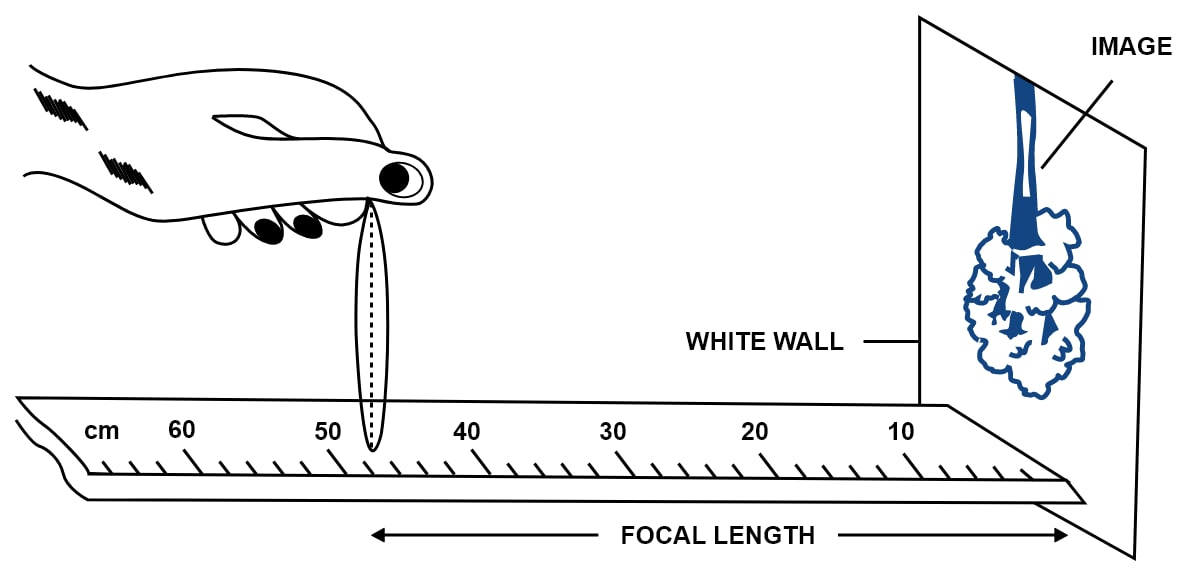#### Question 7

The diagram in figure shows experimental setup for determination of the focal length of a lens using a plane mirror.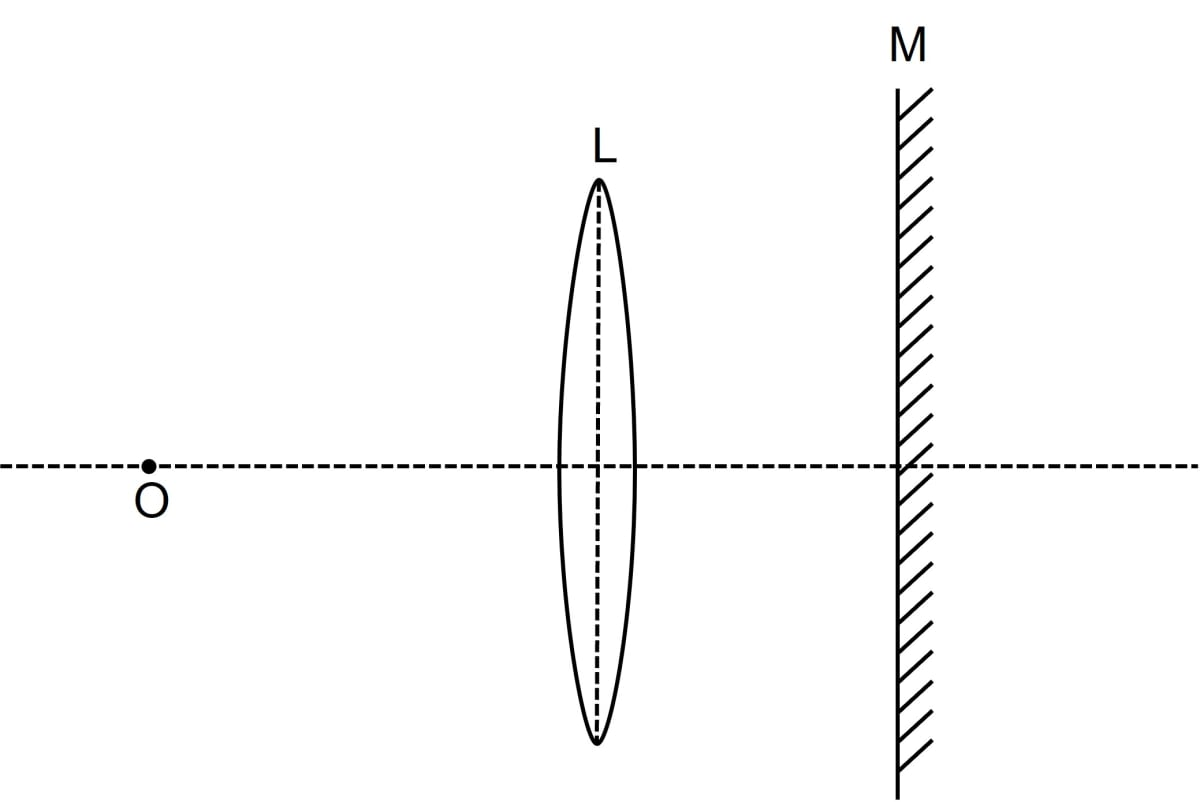(i) Draw two rays from the point O of the object to show the formation of image I at O itself.

(ii) What is the size of the image I?

(iii) State two more characteristics of the image I.

(iv) Name the distance of the object O from the optical centre of the lens.

(v) To what point will the rays return if the mirror is moved away from the lens by a distance equal to the focal length of the lens?

(i) Below ray diagram shows the formation of image I at O itself: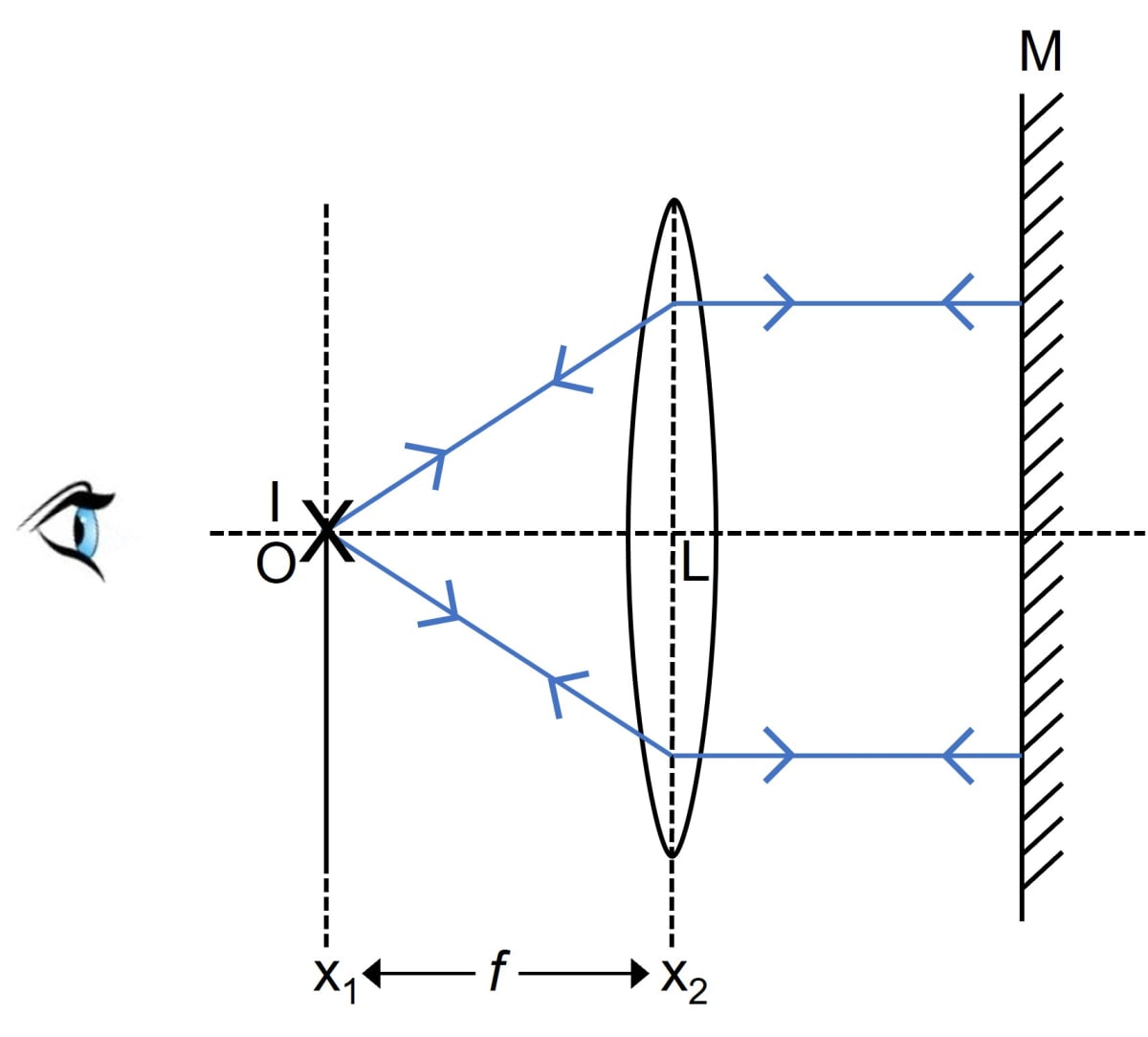(ii) The size of the image is same as that of the object O.

(iii) The image I is inverted and real.

(iv) The distance of object O from the optical centre of the lens will be equal to the focal length of the lens.

(v) The position of plane mirror relative to the lens does not affect the position of image as long as rays fall normally on the plane mirror M.

#### Question 8

Describe how you would determine the focal length of a converging lens, using a plane mirror and one pin. Draw a ray diagram to illustrate your answer.

The equipments required to determine the focal length by using a plane mirror are —

1. A vertical stand
2. A plane mirror
3. A lens
4. A pin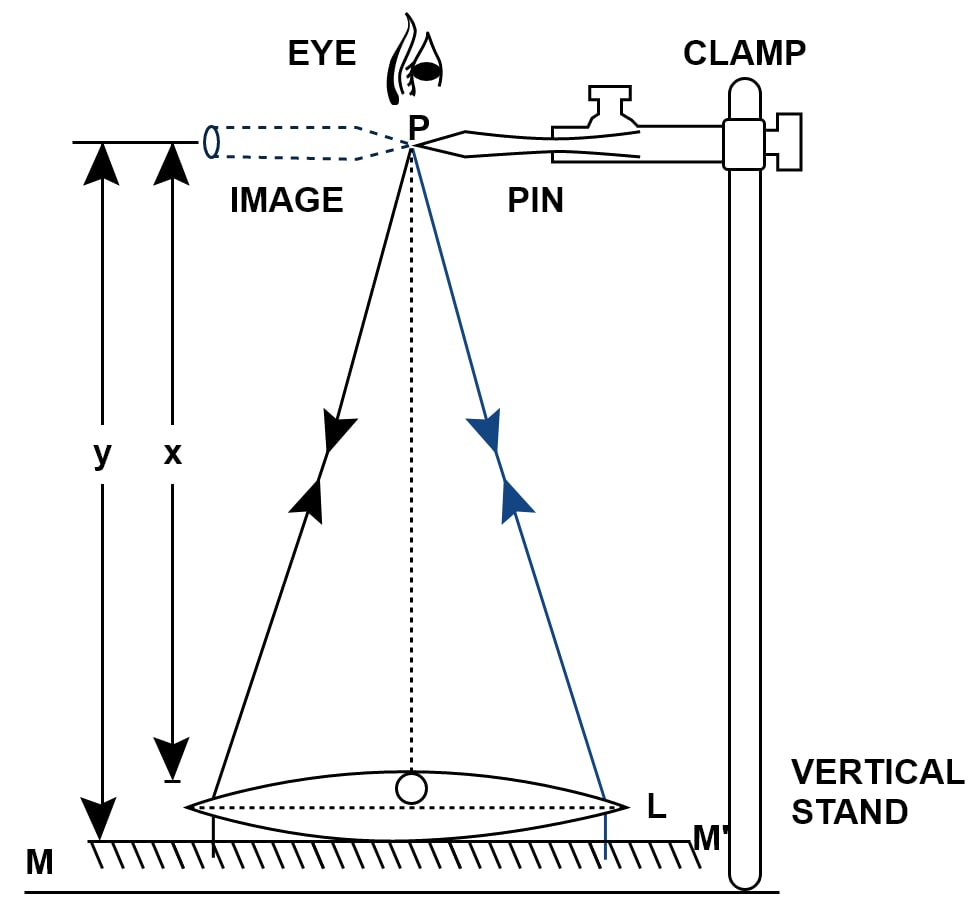(i) We place the lens L horizontally on a plane mirror MM’. Set the pin P in the clamp, in such a way that the tip of the pin is vertically above the centre O of the lens and is perfectly horizontal.

(ii) Set the height of the pin till it has no parallax (i.e., if the pin and its image shift together) so that its inverted image as seen, vertically above the pin.

(ii) Now we measure the distance x of the pin P from the lens and the distance y of the pin from the mirror, using a metre rule and a plumb line.

(iv) Now we calculate the average of the two distances. This gives the focal length of the lens, i.e.,

$f = \dfrac{(x + y)}{2}$

#### Question 9

How will you differentiate between a convex and a concave lens by looking at (i) a distant object and (ii) a printed page?

(i) On seeing a distant object through the lens, if its inverted image is seen, the lens is convex and if the upright image is seen, the lens is concave.

(ii) On keeping the lens near a printed page, if letters appear magnified, the lens is convex and if the letters appear diminished, the lens is concave.

## Multiple Choice Type

#### Question 1

A magnifying glass forms —

1. A real and diminished image
2. A real and magnified image
3. A virtual and magnified image ✓
4. A virtual and diminished image

A magnifying glass forms a virtual and magnified image.

#### Question 2

The maximum magnifying power of a convex lens of focal length 5 cm can be —

1. 25
2. 10
3. 1
4. 6 ✓

As we know,

$m = 1 + \dfrac{D}{f}$

Given,

f = +5 cm

D is the distance of the distinct vision, D = 25 cm

Substituting the values in the formula we get,

$m = 1 + \dfrac{25}{5} \\[0.5em] m = 1 + 5 \\[0.5em] m = 6 \\[0.5em]$

The maximum magnifying power of a convex lens of focal length 5 cm is 6.